## 1 Introduction

For $$d\in \mathbb {N}^+$$, let $$\Lambda \subset \mathbb {R}^d$$ denote a full rank lattice, and let $${\text {Covol}}(\Lambda )>0$$ denote its covolume. Let $$\textbf{A}\in \mathbb {R}^d$$. Consider the function $$N_{d;\Lambda ,\textbf{A}}: [0,\infty )\rightarrow \mathbb {N}^+$$ given by

\begin{aligned} N_{d;\Lambda ,\textbf{A}}(\Sigma ) = \# ([\Lambda +\textbf{A}] \cap \Sigma \mathbb {B}^d) = \# \{\textbf{k}\in \Lambda : |\textbf{k}+\textbf{A}| \le \Sigma \} , \end{aligned}
(1)

where $$\mathbb {B}^d \subset \mathbb {R}^d$$ is the closed unit ball. (For $$\Sigma <0$$, we define $$N_{d;\Lambda ,\textbf{A}}(\Sigma )=0$$.) It is geometrically clear that $${N_{d;\Lambda ,\textbf{A}}(\Sigma )} = \Sigma ^d {\text {Vol}} \mathbb {B}^d {\text {Covol}}(\Lambda )^{-1} + O(\Sigma ^{d-1})$$, as Gauss observed. Let $${N_{d;\Lambda } = N_{d;\Lambda ,\textbf{0}}}$$. The Gauss d-ellipsoid problem (d-sphere problem when $$\Lambda =\mathbb {Z}^d$$) is to find – in the form of a polynomial bound (possibly with logarithmic corrections) – the size of the error $${N_{d;\Lambda }(\Sigma )} - \Sigma ^d {\text {Vol}} \mathbb {B}^d {\text {Covol}}(\Lambda )^{-1}$$. The $$d=2$$ case is known simply as the Gauss ellipse (or circle) problem, and the $$d=3$$ case is known as the Gauss ellipsoid (or sphere) problem. For $$d=2$$, it is conjectured that

\begin{aligned} N_{2;\Lambda }(\Sigma ) = \pi {\text {Covol}}(\Lambda )^{-1} \Sigma ^2 + O( \Sigma ^\nu ) \end{aligned}
(2)

if $$\nu > 1/2$$. See  for a bibliography. For $$d=3$$, it is conjectured that that

\begin{aligned} N_{3;\Lambda }(\Sigma ) = (4\pi /3) {\text {Covol}}(\Lambda )^{-1} \Sigma ^3 + O( \Sigma ^{\nu }) \end{aligned}
(3)

if $$\nu > 1$$. Progress can be found in a bevy of works, going back to Hardy, Landau, Ramanujan, Sierpińsky, and van der Corput, allowing any $$\nu >3/2$$. At present, the best result for the sphere problem is due to Heath-Brown , who shows that we may take any $$\nu >21/16 =1.3125$$ (and whose proof shows that if the generalized Riemann hypothesis holds then, assuming extraneous errors in the proof are dealt with, we may take any $$\nu > 5/4$$). This built off of previous work of Chamizo and Iwaniec , who showed that Eq. (3) holds for any $$\nu > 29/22 = 1.31818\cdots$$, which in turn was based off of papers of Chen  and Vinogradov  which allowed any $$\nu > 4/3$$. See [1, 6, 7] for related results. For arbitrary $$\Lambda \subset \mathbb {R}^3$$, Krätzel and Nowak [8, 9] established Eq. (3) for $$\nu >74/50$$, this being improved by Müller  to $$\nu >63/43$$ and recently Guo  to $$\nu >231/158\approx 1.46202\cdots$$ (these results holding for more general convex bodies as well). See [12, 13] for sharp results for $$d\ge 5$$. Estimating $$N_{3;\Lambda ,\textbf{A}}$$ is a difficult problem. In this note, we do something much easier: examine some related quantities which, in contrast to $$N_{3,\Lambda ,\textbf{A}}$$, can be estimated sharply.

Let $$N_{d,0;\Lambda ,\textbf{A}}=N_{d;\Lambda ,\textbf{A}}$$ and define, for each $$k\in \mathbb {N}^+$$, $$N_{d,k;\Lambda ,\textbf{A}}(\Sigma ) = \int _0^\Sigma N_{d,k-1;\Lambda ,\textbf{A}}(\sigma )\,\textrm{d}\sigma$$, i.e.

\begin{aligned} \begin{aligned} N_{d,1;\Lambda ,\textbf{A}}(\Sigma )&=\int _0^\Sigma N_{d;\Lambda ,\textbf{A}}(\sigma ) \,\textrm{d}\sigma ,\\ N_{d,2;\Lambda ,\textbf{A}}(\Sigma )&=\int _0^\Sigma \int _0^{\sigma } N_{d;\Lambda ,\textbf{A}}(\sigma ') \,\textrm{d}\sigma ' \,\textrm{d}\sigma ,\\ N_{d,3;\Lambda ,\textbf{A}}(\Sigma )&=\int _0^\Sigma \int _0^\sigma \int _0^{\sigma '} N_{d;\Lambda ,\textbf{A}}(\sigma '') \,\textrm{d}\sigma '' \,\textrm{d}\sigma ' \,\textrm{d}\sigma , \cdots \end{aligned} \end{aligned}
(4)

We will refer to these functions as “iterated integrals” of $${N_{d;\Lambda ,\textbf{A}}}$$. We focus on the case $$d=3$$. Even though it is somewhat straightforward (perhaps modulo relatively unimportant technicalities) to give a direct treatment, the authors do not know of one in the literature. (As we will explain below, Hörmander [14, 15] has a general treatment of such asymptotics, but when applied to the case at hand they fail to be sharp.) Our main motivation is to examine how the large-$$\Sigma$$ asymptotics of the $$N_{3,k;\Lambda ,\textbf{A}}$$ are encoded in the Fourier transform of $$N_{d;\Lambda ,\textbf{A}}$$. All other motivation aside, it seems worthwhile to give a relatively self-contained presentation of the results.

Although we cannot deduce from the results below anything interesting about the asymptotics of $$N_{3;\Lambda ,\textbf{A}}$$ beyond the easy result

\begin{aligned} N_{3;\Lambda ,\textbf{A}} = (4\pi /3) {\text {Covol}}(\Lambda )^{-1}\Sigma ^3 + O(\Sigma ^{3/2+\epsilon }), \end{aligned}
(5)

the asymptotics of $$N_{3,1;\Lambda ,\textbf{A}},N_{3,2;\Lambda ,\textbf{A}},\cdots$$ are suggestive and serve well to illustrate the oscillatory nature of the remainder. The results below are therefore complementary to estimates of the second moment

\begin{aligned} \int _0^\Sigma |N_{3,0;\mathbb {Z}^3}(\sigma )-(4\pi /3)\sigma ^3|^2 \,\textrm{d}\sigma \end{aligned}
(6)

as $$\Sigma \rightarrow \infty$$, such as those found in [16, 17], which give no information regarding the sign of the discrepancy $$N_{3,0;\mathbb {Z}^3}(\Sigma )-(4\pi /3)\Sigma ^3$$.

A closely related notion to the iterated integrals above is that of “spectral Riesz means.” For $$k\in \mathbb {N}$$, the kth Riesz mean of the counting function $$N_{3;\Lambda ,\textbf{A}}$$ is defined as

\begin{aligned} R^k_\Sigma N_{3;\Lambda ,\textbf{A}}(\Sigma ) = k!\Sigma ^{-k} N_{3,k;\Lambda ,\textbf{A}} (\Sigma ) = \int _0^\Sigma \Big ( 1 - \frac{\sigma }{\Sigma }\Big )^k \,\textrm{d}N_{3;\Lambda ,\textbf{A}}(\sigma ) . \end{aligned}
(7)

This is the kth Riesz mean of the eigenvalue counting function for the magnetic Schrödinger operator $$\triangle + 2i \textbf{A}\cdot \nabla + \Vert \textbf{A}\Vert ^2$$ on the flat 3-torus $$\mathbb {T}^3 = \mathbb {R}^3/\Lambda ^*$$, where $$\triangle$$ is the positive semidefinite Laplacian and $$\textbf{A}\cdot \nabla = A_x \partial _x+A_y\partial _y+A_z\partial _z$$. It is a theorem of Hörmander [14, 15] that the kth spectral Riesz mean for any closed Riemannian manifold has an asymptotic expansion in powers of $$\Sigma$$, with an $$O(\Sigma ^{d-k-1})$$ error term. Thus, the kth iterated integral of the eigenvalue counting function has an asymptotic expansion in powers of $$\Sigma$$ with an $$O(\Sigma ^{d-1})$$ error term. This error is of size $$O(\Sigma ^2)$$ in the $$d=3$$ case of interest here. The upshot of the analysis below is an $$O(\Sigma )$$ error for $$k\ge 2$$ and is therefore sharper than these much more general results.

In discussing Weyl remainders, it can be useful  to identify the eigenvalue counting with a tempered distribution, especially since we will be working with the Fourier transform. Specifically, letting $$\mathcal {S}(\mathbb {R}) \subset C^\infty (\mathbb {R})$$ denote the Fréchet space of Schwartz functions, we conflate the function $$N_{d;\Lambda ,\textbf{A}}$$ with the mapping

\begin{aligned} \mathcal {S}(\mathbb {R})\ni \chi \mapsto \int _0^\infty \chi (\sigma ) N_{d;\Lambda ,\textbf{A}}(\sigma ) \,\textrm{d}\sigma \in \mathbb {C}. \end{aligned}
(8)

(It is straightforward to verify that Eq. (8) is continuous and therefore defines a tempered distribution.) Doing so essentially trivializes matters of convergence — convergence holds only after “smearing in $$\Sigma$$,” i.e. in the weak topology generated by the functionals given by integration against the various Schwartz functions. This is not an obstacle to getting some ordinarily convergent formula, as Remark 2.3 indicates, but the smearing might obscure relevant features. This is in particular the case for decay rates as $$\Sigma \rightarrow \infty$$.

We will work with two spaces of generalized functions:

• $$\dot{\mathcal {S}}'(\mathbb {R}^{\ge 0}) \subset \mathcal {S}'(\mathbb {R})$$ is the (linear and topological) subspace consisting of tempered distributions supported on $$[0,\infty )={\mathbb {R}^{\ge 0}}$$, that is the set of tempered distributions $$u\in \mathcal {S}'(\mathbb {R})$$ such that $$u(\chi ) = 0$$ whenever the support of $$\chi$$ is disjoint from $$[0,\infty )$$. (In a much more interesting setting than the 1D setting considered here, Melrose  calls them “supported distributions.”) We endow $${\dot{\mathcal {S}'}(\mathbb {R}^{\ge 0})}$$ with the subspace topology induced by the inclusion

\begin{aligned} \dot{\mathcal {S}}'(\mathbb {R}^{\ge 0})\hookrightarrow \mathcal {S}'(\mathbb {R}), \end{aligned}
(9)

which makes $$\dot{\mathcal {S}}'(\mathbb {R}^{\ge 0})$$ into an LCTVS.

• $$\mathcal {S}'(\mathbb {R}^{\ge 0}) = \dot{\mathcal {S}}(\mathbb {R}^{\ge 0})^*$$ (LCTVS-dual), where $${\dot{\mathcal {S}}(\mathbb {R}^{\ge 0}_\Sigma )}$$ denotes the Fréchet space of all smooth functions $$\chi :[0,\infty )_\Sigma \rightarrow \mathbb {C}$$ which vanish to infinite order at the origin and which decay rapidly as $$\Sigma \rightarrow \infty$$, along with all of their derivatives. (Melrose calls the elements of $$\mathcal {S}'(\mathbb {R}^{\ge 0})$$ “extendable distributions.”)

Some facts about these spaces are reviewed in §A. References for the theory of distributions include [19,20,21,22]. For our purposes, it is not necessary to specify a collection of seminorms generating the topology of $$\mathcal {S}'(\mathbb {R}^{\ge 0})$$ — it suffices to note that $$\mathcal {S}'(\mathbb {R}^{\ge 0})$$ is canonically identifiable with

\begin{aligned} \dot{\mathcal {S}}'(\mathbb {R}^{\ge 0}) / \mathbb {C}[\partial ]\delta = \{ u \bmod \mathbb {C}[\partial ]\delta : u \in \dot{\mathcal {S}}'(\mathbb {R}^{\ge 0})\}, \end{aligned}
(10)

where $$\mathbb {C}[\partial ]\delta$$ denotes the set of linear combinations of a Dirac $$\delta$$-function and its derivatives. Indeed, given any $$u\in {\dot{\mathcal {S}}'(\mathbb {R}^{\ge 0})}$$, so that u is a map $$u:\mathcal {S}(\mathbb {R})\rightarrow \mathbb {C}$$, we can restrict u to $${\dot{\mathcal {S}}(\mathbb {R}^{\ge 0})}$$. This defines an onto linear map

\begin{aligned} \dot{\mathcal {S}}'(\mathbb {R}^{\ge 0})\rightarrow \mathcal {S}'(\mathbb {R}^{\ge 0}) \end{aligned}
(11)

whose kernel consists precisely of $$\mathbb {C}[\partial ] \delta$$. Thus, we have an induced invertible linear map

\begin{aligned} \dot{\mathcal {S}}'(\mathbb {R}^{\ge 0}) / \mathbb {C}[\partial ]\delta \rightarrow \mathcal {S}'(\mathbb {R}^{\ge 0}), \end{aligned}
(12)

and it is a homeomorphism. See [19, Lemma 1.2] for the general case.

We can identify any element of $${\cup _{K\in \mathbb {R}} \langle \Sigma \rangle ^K L^1[0,\infty )_\Sigma }$$ with an element of $${\mathcal {S}'(\mathbb {R}_\Sigma ^{\ge 0})}$$ in the same way as done with ordinary tempered distributions.

So, for each $$d\in \mathbb {N}^+$$, $$k\in \mathbb {N}$$, full rank $$\Lambda \subset \mathbb {R}^d$$, and $$\textbf{A}\in \mathbb {R}^d$$, the functions $$N_{d;\Lambda ,\textbf{A}}$$ and $$N_{3,k;\Lambda ,\textbf{A}}$$ can be interpreted as elements of $${\dot{\mathcal {S}}'(\mathbb {R}^{\ge 0})}$$ or $$\mathcal {S}'(\mathbb {R}^{\ge 0})$$. (A clash of notational conventions will lead to us being inconsistent with regards to factors of the Heaviside step function

\begin{aligned} \Theta :\mathbb {R}\rightarrow \{0,1\}, \quad \Theta (\sigma ) = 1_{\sigma \ge 0}. \end{aligned}
(13)

When considering $$N_{3,k;\Lambda ,\textbf{A}}$$ as a tempered distribution, we must write the step functions explicitly, but when we are working in $${\dot{\mathcal {S}}'(\mathbb {R}^{\ge 0})}$$ we will typically omit them.)

### Remark 1

We follow the notational convention of Melrose in using overdots, e.g. ‘$$\mathcal {S}'(\mathbb {R}^{\ge 0})$$’ to denote the dual of $$\dot{\mathcal {S}}(\mathbb {R}^{\ge 0})$$ and ‘$$\dot{\mathcal {S}}'(\mathbb {R}^{\ge 0})$$’ to denote the dual of $$\mathcal {S}(\mathbb {R}^{\ge 0})$$. So, according to this convention, the ‘$$'$$’ should not be read as dualization. We will mostly drop the ‘$$\bmod \,\mathbb {C}[\partial ]\delta$$’ that should be written when specifying elements of $$\dot{\mathcal {S}}'(\mathbb {R}^{\ge 0}) / \mathbb {C}[\partial ]\delta$$. Hence, we will notationally conflate extendable distributions with supported distributions extending them. In addition, as discussed above, we will make ample use of conventional abuses of notation allowing the identification of locally integrable, polynomially growing functions with tempered distributions. Regarding formal variables, when we write ‘$$N_{3;\Lambda }(\Sigma )\in \mathcal {S}'(\mathbb {R}_\Sigma )$$’ (or anything similar), we mean that $$N_{3;\Lambda }$$ is identifiable with an element of $$\mathcal {S}'(\mathbb {R})$$, with $$\Sigma$$ playing a formal role, notationally speaking. The (optional) subscripts just declare which variable is being integrated against where such disambiguation is felt to be useful.

In the latter half of our presentation, §4 and §5, we turn to the toroidal half-wave trace. Because $$N_{3;\Lambda ,\textbf{A}}(\Sigma )$$ is nondecreasing, its Fourier transform must be defined in the sense of Schwartz; explicitly, $$\mathcal {F}N_{3;\Lambda ,\textbf{A}}: \mathcal {S}(\mathbb {R})\rightarrow \mathbb {C}$$ is given by

\begin{aligned} \mathcal {F}N_{3;\Lambda ,\textbf{A}}( \chi ) = \int _0^\infty N_{3;\Lambda ,\textbf{A}}(\Sigma ) \mathcal {F}\chi (\Sigma ) \,\textrm{d}\Sigma \end{aligned}
(14)

for any $$\chi \in \mathcal {S}(\mathbb {R})$$, where our conventions in defining $$\mathcal {F}:\mathcal {S}(\mathbb {R})\rightarrow \mathcal {S}(\mathbb {R})$$ are

\begin{aligned} \quad \mathcal {F}\chi (\sigma ) = \int _{-\infty }^{+\infty } e^{-i\tau \sigma } \chi (\tau ) \,\textrm{d}\tau . \end{aligned}
(15)

(We will use $$\tau$$ to denote the Fourier dual variable to $$\Sigma ,\sigma$$.) Equation (14) is the Fourier transform with respect to $$\Sigma$$, as opposed to the coordinate $$\Sigma ^2$$, which would correspond to the heat kernel rather than the wave kernel. While $$\mathcal {F}N_{3;\Lambda ,\textbf{A}}$$ is a well-defined tempered distribution, it is not a function — it has singularities, and we will keep careful track of them. The Laplace transform of $$N_{3;\Lambda ,\textbf{A}}(\Sigma )$$ in $$\Sigma ^2$$ (which is well-defined as an improper integral) is essentially a power of a Jacobi theta function, therefore an automorphic form after analytic continuation, and this entails that the Fourier transform of $$N_{3;\Lambda ,\textbf{A}}(\Sigma )$$ in $$\Sigma ^2$$ (the distributional boundary value of a modular form at the real axis) has full singular support. It is a tempered distribution, but it is nowhere locally a function. In contrast, $$\mathcal {F}N_{3;\Lambda ,\textbf{A}}(\tau )$$ has an isolated singularity at $$\tau = 0$$ (and actually has a Laurent series there), and, as we will see, is in fact a function except at a discrete set of times, around each of which $$\mathcal {F}N_{3;\Lambda ,\textbf{A}}(\tau )$$ has a Laurent series:

### Theorem 1.1

Setting $$\mu _{3;\Lambda ,\textbf{A}}(\Sigma ) = \sum _{{\varvec{\Sigma }} \in \Lambda } \delta (\Sigma -|\varvec{\Sigma }+\textbf{A}|) \in \mathcal {S}'(\mathbb {R}_\Sigma )$$, the toroidal half-wave trace $$\mathcal {F}\mu _{3;\Lambda ,\textbf{A}}(\tau )\in \mathcal {S}'(\mathbb {R}_\tau )$$ is given by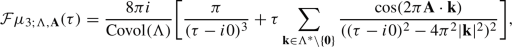(16)

where the sum is unconditionally convergent in $$\mathcal {S}'(\mathbb {R}_\tau )$$.

Integrating this by means of Proposition A.4, we ge get an explicit formula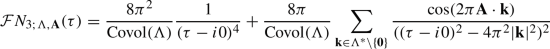(17)

for $$\mathcal {F}N_{3;\Lambda ,\textbf{A}}$$.

The half-wave trace on general compact Riemannian manifolds was studied by Chazarain , Hörmander , and Duistermaat & Guillemin , and together they established the precise singularity structure of the half-wave trace of any closed Riemannian manifold. We will not use this general theory, since we can just compute $$\mathcal {F}N_{3;\Lambda ,\textbf{A}}(\tau )$$ explicitly, but the singularity structure in Eq. (16) is in accord with these more general results. That $$\mathcal {F}N_{3;\Lambda ,\textbf{A}}(\tau )$$ has an isolated singularity (of known form) at the origin is useful, because it allows us to separate the polynomial growth of $$N_{3;\Lambda ,\textbf{A}}(\Sigma )$$ as $$\Sigma \rightarrow \infty$$ (coming from the pole of the Fourier transform at the origin) and an oscillatory remainder, coming from the rest. From this perspective, the additional result needed to deduce sharp asymptotics for the $$N_{d,k;\Lambda ,\textbf{A}}$$ (for sufficiently large k) is global quantitative control on the half-wave trace, with a polynomial rate of growth (where the meaning of “sufficiently large” is determined by the degree of the rate of growth).

Via the Poisson summation formula, we can compute out the oscillatory contribution to $$N_{d,0;\Lambda ,\textbf{A}}$$ exactly, for every $$d\ge 1$$: it is an infinite sum of Bessel functions, yielding a formula for $$N_{d;\Lambda ,\textbf{A}}(\Sigma )$$ generalizing (in a weak, distributional sense) the well-known formula for $$N_{2;\mathbb {Z}^2}(\Sigma )$$ — cf. [25, §4.4]. While we cannot estimate the size of this oscillatory term precisely (a problem familiar from the Gauss circle problem, which is still open, despite the analogous formula being known for a century), the situation changes upon integration in $$\Sigma$$, since upon passing to the Fourier transform this corresponds to a weighting by $$1/\tau$$, which improves decay (away from the origin) as $$\tau \rightarrow \pm \infty$$. Hence, after integrating, we can well-estimate the oscillatory term – elementarily too, at least in the $$d=3$$ case we restrict attention to. However, the division by $$\tau$$ makes more severe the singularity of the half-wave trace at the origin. Applying $$\mathcal {F}^{-1}$$ then yields new polynomial terms. The end result is:

### Theorem 1.2

For each $$k=0,1,2,3,\ldots$$,

\begin{aligned} N_{3,k;\Lambda ,\textbf{A}}(\Sigma ) = \frac{8\pi }{(3+k)!} \frac{\Sigma ^{3+k}}{{\text {Covol}}(\Lambda )} + \sum _{m=0}^{k-1} \frac{C_{k-1-m;\Lambda ,\textbf{A}}}{m!} \Sigma ^m + o_{k;\Lambda ,\textbf{A}}(\Sigma ) \end{aligned}
(18)

as an element of $$\dot{\mathcal {S}}'(\mathbb {R}^{\ge 0})$$, where $$\{C_{j;\Lambda ,\textbf{A}}\}_{j=0}^\infty \subset \mathbb {R}$$ is defined by (the absolutely convergent series)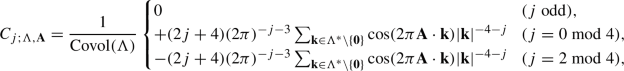(19)

and $$o_{k;\Lambda ,\textbf{A}} \in \dot{\mathcal {S}}'(\mathbb {R}^{\ge 0})$$ is given by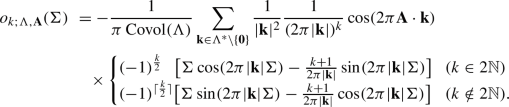(20)

The series in Eq. (20) is unconditionally summable in $$\dot{\mathcal {S}}'(\mathbb {R}^{\ge 0})$$.

If $$k\ge 2$$, then Eq. (18) holds in the ordinary sense, for each $$\Sigma \ge 0$$ (with the series in Eq. (20) ordinarily absolutely convergent). Moreover, if $$k\ge 2$$, $$o_{k;\Lambda ,\textbf{A}}:[0,\infty ) \rightarrow \mathbb {R}$$ is continuous. It vanishes at the origin, and, for any compact $$K\subset {\mathbb {R}^{\ge 0}}$$, the series in Eq. (20), restricted to K, converges uniformly to it.

### Corollary 1.3

As $$\Sigma \rightarrow \infty$$,

\begin{aligned} N_{3,1;\Lambda ,\textbf{A}}(\Sigma ) = \frac{\pi }{3} \frac{\Sigma ^4}{{\text {Covol}}(\Lambda )} + O_{\Lambda ,\textbf{A}}(\Sigma \log \Sigma ) \end{aligned}
(21)

holds.

### Corollary 1.4

For each $$k\ge 2$$,

\begin{aligned} N_{3,k;\Lambda ,\textbf{A}}(\Sigma ) = \frac{8\pi }{(3+k)!} \frac{\Sigma ^{3+k}}{{\text {Covol}}(\Lambda )} + \sum _{m=2}^{k-1} \frac{C_{k-1-m;\Lambda ,\textbf{A}}}{m!} \Sigma ^m + O_{k,\Lambda ,\textbf{A}}(\Sigma ) \end{aligned}
(22)

for all $$\Sigma \ge 0$$.

The subscripts on the big-O’s denote that the bounds depend on the listed parameters in some unexamined way. Below, we will leave this dependence implicit.

Finally, we check that there are no serious cancellations in Eq. (20):

### Theorem 1.5

For no integer $$k \ge 1$$ does there exist an $$\alpha =\alpha _{\textbf{A},\Lambda ,k}\in \mathbb {R}$$ and $$\epsilon >0$$ such that

\begin{aligned} N_{3,k;\Lambda ,\textbf{A}}(\Sigma ) = \frac{8\pi }{(3+k)!} \frac{\Sigma ^{3+k}}{{\text {Covol}}(\Lambda )} + \sum _{m=2}^{k-1} \frac{C_{k-1-m;\Lambda ,\textbf{A}}}{m!} \Sigma ^m + \alpha \Sigma + O(\Sigma ^{1-\epsilon }) \end{aligned}

as $$\Sigma \rightarrow \infty$$.

See Fig. 1 for an illustration of Theorem 1.2, Corollary 1.4, Theorem 1.5 in the instructive case $$k=4$$, $$\Lambda =\mathbb {Z}^3$$, $$\textbf{A}=0$$. The oscillatory character of $$N_{3,4;\mathbb {Z}^3}(\Sigma ) - (\pi /630) \Sigma ^7 - (C_{0;\Lambda }/6)\Sigma ^3-C_{2;\Lambda } \Sigma$$ is clearly visible, as is the linear growth rate of the amplitudes of the oscillatory remainder.

The computation used below to prove the first of these results is very standard — it is just a (somewhat nondirect) application of the Poisson summation formula, keeping track of the possible singularity at the origin. It can be understood in terms of the “method of images” for solving PDE on $$\mathbb {T}^d$$, but this just amounts to an application of the Poisson summation formula anyways. A brisk presentation of the core of the argument in the case $$\Lambda =\mathbb {Z}^3$$, $$\textbf{A}=0$$ can be found in [3, 25], and a presentation of van der Corput’s version can be found in . The case of a general lattice or nonzero shift does not require any new ideas. (To compare our treatment to, say, that in , we provide a summation formula for $${N_{3,0;\mathbb {Z}^3}}$$ in $${\dot{\mathcal {S}}'(\mathbb {R}^{\ge 0})}$$, not just in $${\mathcal {S}'(\mathbb {R}^{\ge 0})}$$, this being useful in the proof of Theorem 1.1.) The results for $$k>0$$ require in our presentation a secondary technical argument in §3 (though after some smoothing in $$\Sigma$$ this can be replaced by an appeal to the precise amount of regularity in $$\varvec{\Sigma }$$ required in [3, Lemma 2.1][25, Corollary 4.8]). The slightly technical point here is that in the traditional presentation of these sorts of computations, one wants to apply the Poisson summation formula to the function $$f_\Sigma (\varvec{\Sigma })=(\Sigma -|\varvec{\Sigma }|)_+^k \in C^0(\mathbb {R}^d_{\varvec{\Sigma }})$$, which is not smooth and therefore not Schwartz. Once can appeal to [27, Chp. VII, Cor. 2.6] in order to justify the application, but this requires first computing out $$\mathcal {F}f_\Sigma$$. The computation of this Fourier transform is analogous to the computation in §3.

Corollary 1.3 is extracted from Theorem 1.2 in §4 via a very elementary smoothing argument.

We elaborate briefly on how the results above give more precise information regarding spectral Riesz means on flat 3-tori than what is known for general manifolds. Hörmander’s asymptotic expansion for the spectral Riesz means of $$\mathbb {T}^3$$ is contained in Corollary 1.3, Corollary 1.4. The asymptotic expansions in Corollary 1.3 and Corollary 1.4 are more precise, however — besides being completely explicit about what the coefficients in Hörmander’s asymptotic expansion are, the error term is only of size $$O(\Sigma )$$ for $$k\ge 2$$ and $$O(\Sigma \log \Sigma )$$ for $$k=1$$, instead of $$O(\Sigma ^2)$$. Moreover, for $$k\ge 2$$, Theorem 1.2 gives an absolutely convergent trigonometric sum for the error term, which is very particular to the torus. To boot, whereas it is typically not known when Hörmander’s $$O(\Sigma ^2)$$ estimate is sharp, the estimate here is actually sharp, as Theorem 1.5 shows. We think that Corollary 1.4 is interesting in part as a sharp estimate of some spectral Riesz means, and to our knowledge this result is the first sharp estimate of higher Riesz means for some non-Zoll manifolds of dimension $$>1$$. Although we do not carry it out, an analogous computation to the one below gives sharp asymptotics for the “off-diagonal” spectral Riesz means of $$\mathbb {T}^3$$. See [14, 28] for more on spectral Riesz means in general.

Conceptually, the feature of $$\mathbb {T}^3$$ – and flat tori more generally – that allows proving this refined asymptotic expansion is the slow growth of the (say 2-fold regularized) toroidal half-wave trace (as measured in an appropriate sense). Thus, while our proof is partly based on an explicit computation, a similar result will hold on any closed Riemannian manifold on which the half-wave trace grows polynomially with respect to an appropriate Sobolev-type norm measuring negative regularity. See  for a related discussion of Weyl remainders themselves. The detailed analysis of the toroidal half-wave trace found in §5 is difficult to locate in the literature (if it exists, it is unknown to the authors). We use the results in this section to prove Theorem 1.5. We remark in closing that, even in the $$\Lambda =\mathbb {Z}^3,\textbf{A}=0$$ case, the summation formulas [3, Lemma 2.1][25, Corollary 4.8] do not suffice for the proof of Theorem 1.1 (they only determine $$\mathcal {F}\mu _{3;\Lambda ,\textbf{A}}$$ modulo a polynomial), so it is not clear that one can avoid the additional technical details in the treatment below.

## 2 An explicit functional formula for $$N_{d;\Lambda ,\textbf{A}}$$

The Poisson summation formula for $$\Lambda$$ says that

\begin{aligned} \sum _{\varvec{\Sigma }\in [\Lambda +\textbf{A}]} f(\varvec{\Sigma })= & {} \sum _{\varvec{\Sigma }\in \Lambda }f_{\textbf{A}}(\varvec{\Sigma }) = \frac{1}{{\text {Covol}}(\Lambda )} \sum _{\textbf{k}\in \Lambda ^*} \mathcal {F}f_\textbf{A}( 2\pi \textbf{k}) \nonumber \\= & {} \frac{1}{{\text {Covol}}(\Lambda )} \sum _{\textbf{k}\in \Lambda ^*} e^{2\pi i \textbf{k}\cdot \textbf{A}} \mathcal {F}f ( 2\pi \textbf{k}) \end{aligned}
(23)

for all $$f\in \mathcal {S}(\mathbb {R}^d)$$, where $$f_{\textbf{A}}(\varvec{\Sigma })=f(\varvec{\Sigma }+\textbf{A})$$. In other words, both sums converge unconditionally – trivially, in this case, because f and $$\mathcal {F}f$$ are Schwartz – and their limits agree.

Let $$\varvec{\Sigma }= (\sigma _1,\cdots ,\sigma _d)$$ and $$r=(\sigma _1^2+\cdots +\sigma _d^2)^{1/2} = \Vert \varvec{\Sigma }\Vert$$.

Suppose now that f has the form $$f(\sigma _1,\ldots ,\sigma _d)=F(r)$$ for some $$F\in \mathcal {S}(\mathbb {R})$$ whose odd-order derivatives all vanish at zero. For example, any

\begin{aligned} F \in \dot{\mathcal {S}} (\mathbb {R}^{\ge 0}) = \dot{C}^\infty (\mathbb {R}^{\ge 0})\cap \mathcal {S}(\mathbb {R}) \end{aligned}
(24)

has this property, as does any Schwartz function that is constant in some neighborhood of the origin.

For such F, f is actually Schwartz, so the Poisson summation formula applies, yielding

\begin{aligned} \sum _{\varvec{\Sigma }\in [\Lambda +\textbf{A}]} F(|\varvec{\Sigma }|)&= \frac{1}{{\text {Covol}}(\Lambda )} \sum _{\textbf{k}\in \Lambda ^*} e^{2\pi i \textbf{k}\cdot \textbf{A}} \mathcal {F}f ( 2\pi \textbf{k}) \end{aligned}
(25)
\begin{aligned}&= \frac{1}{{\text {Covol}}(\Lambda )} \sum _{\textbf{k}\in \Lambda ^*} e^{2\pi i \textbf{k}\cdot \textbf{A}} \int _{0}^\infty F(r) r^{d-1} \int _{\mathbb {S}^{d-1}} e^{-2\pi i r\textbf{k}\cdot \varvec{\theta }} \,\textrm{d}\!{\text {Area}}_{\mathbb {S}^{d-1}} (\varvec{\theta }) \,\textrm{d}r. \end{aligned}
(26)

In the expression above is a standard form of the Bessel function of the first kind:

\begin{aligned} \int _{\mathbb {S}^{d-1}} e^{i \textbf{w}\cdot \varvec{\theta }} \,\textrm{d}\! {\text {Area}}_{\mathbb {S}^{d-1}} (\varvec{\theta }) = {\left\{ \begin{array}{ll} (2\pi )^{\nu + 1 } |\textbf{w}|^{-\nu } J_\nu (|\textbf{w}|) &{} (\textbf{w}\ne 0), \\ {\text {Area}}(\mathbb {S}^{d-1}) &{} (\textbf{w}= 0), \end{array}\right. } \end{aligned}
(27)

where $$\nu = d/2-1$$. See [30, pg. 198][27, pg. 154]. Substituting this into Eq. (26),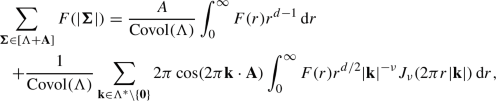(28)

where $$A={\text {Area}}(\mathbb {S}^{d-1})$$ and we used the symmetry of $$\Lambda ^*$$ to replace the $$\exp (2\pi i \textbf{k}\cdot \textbf{A})$$ with $$\cos (2\pi \textbf{k}\cdot \textbf{A})$$.

Note that the formal series $$\sum _{\varvec{\Sigma }\in \Lambda } \delta (\Sigma - |\varvec{\Sigma }+\textbf{A}|) \in \mathcal {S}'(\mathbb {R}_\Sigma )^{\Lambda }$$ is unconditionally summable to a tempered distribution (which is in fact a Borel measure), which we denote $$\Delta (\Sigma )=\sum _{\varvec{\Sigma }\in \Lambda } \delta (\Sigma - |\varvec{\Sigma }+\textbf{A}|)$$. The left-hand side of Eq. (28) can therefore be written as follows:

\begin{aligned} \sum _{\varvec{\Sigma }\in [\Lambda +\textbf{A}]} F(| \varvec{\Sigma }|) = \int _{-\infty }^{+\infty } F(\Sigma ) \sum _{\varvec{\Sigma }\in \Lambda } \delta (\Sigma - |\varvec{\Sigma }+\textbf{A}|) \,\textrm{d}\Sigma = \Delta (F), \end{aligned}
(29)

where the integral is formal. Similarly – letting, for $$\textbf{k}\ne 0$$, $$\mathcal {J}_{\textbf{k}} \in \mathcal {S}'(\mathbb {R})$$ denote the tempered distribution $$\chi \mapsto \int _{\mathbb {R}} \chi (\Sigma ) \mathcal {J}_{\textbf{k}}(\Sigma ) \,\textrm{d}\Sigma$$ given by integration against the function

\begin{aligned} \mathcal {J}_{\textbf{k}}(\Sigma ) = {\left\{ \begin{array}{ll} 2\pi \Sigma ^{d/2} |\textbf{k}|^{-\nu } J_\nu (2\pi |\textbf{k}|\Sigma ) &{} (\Sigma >0) \\ 0 &{} (\Sigma \le 0), \end{array}\right. } \end{aligned}
(30)

(which we denote $$\mathcal {J}_{\textbf{k}}(\Sigma ) = 2\pi \Theta (\Sigma ) \Sigma ^{d/2} |\textbf{k}|^{-\nu } J_\nu (2\pi \Sigma |\textbf{k}|)$$) – the summand on the right-hand side of Eq. (28) is $$\cos (2\pi i \textbf{k}\cdot \textbf{A})\mathcal {J}_{\textbf{k}}(F)$$. (Note that the subscript ‘$$\textbf{k}$$’ in $$\mathcal {J}_{\textbf{k}}$$ is not related to the subscript ‘$$\nu$$’ in $$J_\nu$$.)

The formal series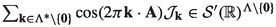is unconditionally summable in $$\mathcal {S}'(\mathbb {R}^{\ge 0})$$, as an integration-by-parts argument shows. The identities above show that(31)

for all F as above. So, we have arrived at [25, Theorem 4.6]:

### Proposition 2.1

As elements of $$\mathcal {S}'(\mathbb {R}^{\ge 0}_\Sigma )$$,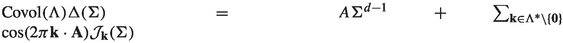.

We will need to pay attention to Dirac terms, but first we observe the following, which is suggested by formally integrating the identity in Proposition 2.1:

### Proposition 2.2

As elements of $$\mathcal {S}'(\mathbb {R}^{\ge 0}_\Sigma )$$, we have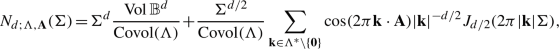(32)

where the sum is unconditionally convergent in $$\mathcal {S}'(\mathbb {R}^{\ge 0}_\Sigma )$$.

### Proof

We only need to rigorously justify the formal integration. We first check that the left-hand and right-hand sides of Eq. (32), considered as elements of $$\mathcal {S}'(\mathbb {R}^{\ge 0})$$, have the same derivative. We observe:

1. (I)

$$\partial N_{d;\Lambda ,\textbf{A}}(\Sigma ) = \Delta (\Sigma )$$ when both sides are considered as elements of $$\mathcal {S}'(\mathbb {R})$$, so this continues to hold when both sides are continued as elements of $$\mathcal {S}'(\mathbb {R}^{\ge 0})$$, and

2. (II)

the sum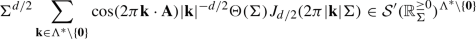(33)

is unconditionally convergent in $$\mathcal {S}'(\mathbb {R}^{\ge 0})$$ by an integration-by-parts argument, and the derivative of the extendable distribution in Eq. (33) is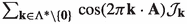.

So, indeed, the two sides of Eq. (32) have the same derivative in $$\mathcal {S}'(\mathbb {R}^{\ge 0})$$. This is equivalent to saying that, when interpreted as elements of $${\dot{\mathcal {S}}'(\mathbb {R}^{\ge 0})}$$, they have the same derivative modulo $$\mathbb {C}[\partial ]\delta$$.

By Proposition A.1, we conclude that, as an element of $${\mathcal {S}'(\mathbb {R}^{\ge 0}_\Sigma )}$$,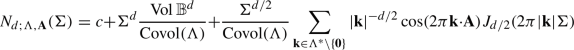(34)

for some constant $$c = c_{\Lambda ,\textbf{A}}\in \mathbb {C}$$, which we have to show is zero. (We are omitting the $$\Theta (\Sigma )$$ in Eq. (34).)

For $$\chi \in C_{\textrm{c}}^\infty (\mathbb {R})$$ that is constant in a neighborhood of the origin, we calculate $$N_{d;\Lambda ,\textbf{A}}(\partial \chi ) = \int _0^\infty N_{d;\Lambda ,\textbf{A}}(\Sigma )\chi '(\Sigma ) \,\textrm{d}\Sigma$$ in two ways:

• Since $$\partial N_{d;\Lambda ,\textbf{A}}(\Sigma ) = \Delta (\Sigma )$$ as elements of $$\mathcal {S}(\mathbb {R})$$, $$N_{d;\Lambda ,\textbf{A}}(\partial \chi )=-\Delta (\chi )$$. By Eq. (28),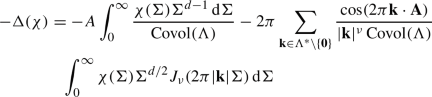(35)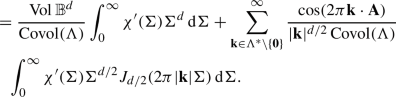(36)
• Applying Eq. (34), which we can do because $$N_{d;\Lambda ,\textbf{A}}(\partial \chi ) = N_{d;\Lambda ,\textbf{A}}(\Theta \partial \chi )$$ and $$\Theta \partial \chi \in \dot{\mathcal {S}}(\mathbb {R}^{\ge 0})$$, yields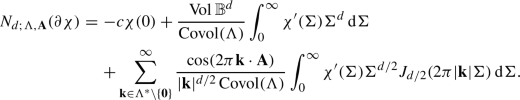(37)

Comparing Eqs. (36) and (37), we deduce that $$c\int _0^\infty \chi '(\Sigma ) \,\textrm{d}\Sigma = -c \chi (0) =0$$. Since we can choose $$\chi$$ as above that is nonzero at zero, we conclude that $$c=0$$. $$\square$$

### Remark 2.3

When $$d=2$$, $$\sum _{n=1}^\infty r_2(n) n^{-1/2} J_1(2\pi \sqrt{n} \Sigma )$$ converges (conditionally) for $$\Sigma >0$$ with $$\Sigma ^2 \notin \mathbb {Z}$$ (as shown by Hardy). If $$d\ge 3$$ then there does not exist any $$\Sigma >0$$ such that the sum on the right-hand side of Eq. (32) converges in the ordinary sense, hence it must be interpreted “distributionally:” given any Schwartz function $$\chi \in \mathcal {S}(\mathbb {R})$$,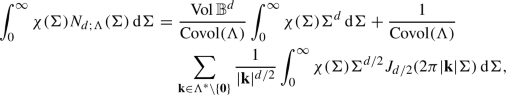(38)

where the sum on the right-hand side is absolutely convergent. For example, since $$N_{d;\mathbb {Z}^d}$$ is constant on intervals of the form $$[\sqrt{n}, \sqrt{n+1})$$ for $$n\in \mathbb {N}$$, choosing $$\chi \in C_{\textrm{c}}^\infty (\mathbb {R})$$ supported in $$[\sqrt{n}, \sqrt{n+1})$$ with $$\int _\mathbb {R}\chi (\Sigma ) \,\textrm{d}\Sigma = 1$$,

\begin{aligned} N_{d;\mathbb {Z}^d}(\sqrt{n}) = {\text {Vol}} \mathbb {B}^{d}\int _0^\infty \chi (\Sigma ) \Sigma ^d \,\textrm{d}\Sigma + \sum _{n=1}^\infty \frac{r_d(n)}{n^{d/4}} \int _0^\infty \chi (\Sigma ) \Sigma ^{d/2} J_{d/2}(2\pi \sqrt{n} \Sigma ) \,\textrm{d}\Sigma , \end{aligned}
(39)

where $$r_d(n) = \{\textbf{k}\in \mathbb {Z}^d: |\textbf{k}| = n\}$$.

Whenever $$d \in 2\mathbb {N}+1$$, the Bessel function $$J_{d/2}(z)$$ can be written as a linear combination of trigonometric functions whose coefficients are Laurent polynomials in z. For $$d=3$$, we have

\begin{aligned} J_{3/2}(z) = \sqrt{\frac{2}{\pi z}}\left( -\cos (z) + \frac{1}{z} \sin (z)\right) \end{aligned}
(40)

for all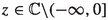. Substituting this in to Eq. (32), we get:

### Proposition 2.4

As an element of $$\mathcal {S}'(\mathbb {R}^{\ge 0}_\Sigma )$$, $$N_{3;\Lambda ,\textbf{A}}(\Sigma )$$ is given by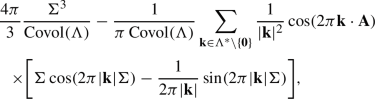(41)

where the sum on the right-hand side is (unconditionally) convergent in $$\mathcal {S}'(\mathbb {R}^{\ge 0}_\Sigma )$$.

## 3 An explicit formula for $$N_{3,k;\Lambda ,\textbf{A}}$$, $$k\ge 1$$

We now proceed to iterate the formal integration leading us from Proposition 2.1 to Proposition 2.2. We have not, however, yet proven that the series on the right-hand side of Eq. (32) converges unconditionally in $$\dot{\mathcal {S}}'(\mathbb {R}^{\ge 0})$$, i.e. in $$\mathcal {S}'(\mathbb {R})$$ (after inserting $$\Theta$$’s) (we only noted that – by integration-by-parts – they converge unconditionally in $$\mathcal {S}'(\mathbb {R}^{\ge 0}) \cong \dot{\mathcal {S}}'(\mathbb {R}^{\ge 0})/\mathbb {C}[\partial ]\delta$$), and the computations in the previous section, leading up to Eq. (28), were only done for Schwartz functions $$F \in \mathcal {S}(\mathbb {R})$$ that are the sum of an even function and an element of $$\dot{\mathcal {S}}(\mathbb {R}^{\ge 0})$$. For proving Proposition 2.2, this sufficed, but for repeated integrations we need to allow arbitrary Schwartz F.

For each $$k\in \mathbb {N}$$, let $$\partial ^{-k}: \dot{\mathcal {S}}'(\mathbb {R}^{\ge 0}) \rightarrow \dot{\mathcal {S}}'(\mathbb {R}^{\ge 0})$$ denote the well-defined set-theoretic inverse of $$\partial ^k$$, i.e. the kth power of the operator $$\partial ^{-1}$$ defined in Eq. (137). Heuristically, $$\partial ^{-k} \chi$$ is given by k-fold iterated integrations of $$\chi$$ from $$0^+$$ and this holds literally when applied to integrable functions, and consequently

\begin{aligned} \partial ^{-k} N_{3;\Lambda ,\textbf{A}}(\Sigma ) = N_{3,k;\Lambda ,\textbf{A}}(\Sigma ), \end{aligned}
(42)

where both sides are interpreted as elements of $${\dot{\mathcal {S}}'(\mathbb {R}^{\ge 0})}$$.

As a preliminary step in computing $$N_{3,k;\Lambda ,\textbf{A}}$$ via Eq. (42), we define $$\{\tilde{N}_{3,k;\Lambda ,\textbf{A}} \}_{k\in \mathbb {N}}\subset \mathcal {S}'(\mathbb {R}^{\ge 0})$$ such that

• $$\tilde{N}_{3,0;\Lambda ,\textbf{A}} = N_{3,0;\Lambda ,\textbf{A}}$$ in $$\mathcal {S}'(\mathbb {R}^{\ge 0})$$,

• and

\begin{aligned} \tilde{N}_{3,0;\Lambda ,\textbf{A}}(\Sigma ) =\partial ^{k} \tilde{N}_{3,k;\Lambda ,\textbf{A}}(\Sigma ), \end{aligned}
(43)

also in $$\mathcal {S}'(\mathbb {R}^{\ge 0})$$. We will check that the formal series defining $$\tilde{N}_{3,k;\Lambda ,\textbf{A}}$$ converges unconditionally in $${\dot{\mathcal {S}}'(\mathbb {R}^{\ge 0})}$$ for $$k\ge 2$$, and by differentiating we will conclude the same for $$k=0,1$$, hence essentially proving that the series on the right-hand side of Proposition 2.1 converges absolutely in some negative regularity Sobolev space.

This will allow us to interpret Eq. (43) as an identity in $$\dot{\mathcal {S}}'(\mathbb {R}^{\ge 0})$$.

Given now that $$\tilde{N}_{3,0;\Lambda ,\textbf{A}}$$ is a well-defined element of $$\dot{\mathcal {S}}'(\mathbb {R}^{\ge 0})$$, the fact that $$\tilde{N}_{3,0;\Lambda ,\textbf{A}} = N_{3,0;\Lambda ,\textbf{A}}$$ in $$\mathcal {S}'(\mathbb {R}^{\ge 0})$$ means that

\begin{aligned} \tilde{N}_{3,0;\Lambda ,\textbf{A}}(\Sigma ) = P(\partial ) \delta (\Sigma ) + N_{3,0;\Lambda ,\textbf{A}}(\Sigma ) \end{aligned}
(44)

in $$\dot{\mathcal {S}}(\mathbb {R}^{\ge 0}_\Sigma )$$ for some polynomial $$P=P_{\Lambda ,\textbf{A}}$$. Equation (43) then determines $$\tilde{N}_{3,k;\Lambda ,\textbf{A}}$$ in terms of $$N_{3,0;\Lambda ,\textbf{A}}$$ modulo polynomials and derivatives of Dirac $$\delta$$-functions (the results of integrating $$\mathbb {C}[\partial ]\delta$$).

It will turn out that we defined $$\tilde{N}_{3,k;\Lambda ,\textbf{A}}$$ so that, as elements of $$\dot{\mathcal {S}}'(\mathbb {R}^{\ge 0})$$,

\begin{aligned} \tilde{N}_{3,k;\Lambda ,\textbf{A}} = N_{3,k;\Lambda ,\textbf{A}}, \end{aligned}
(45)

meaning that $$P=0$$. This will be proven by checking that $$\lim _{\Sigma \rightarrow 0^+} \tilde{N}_{3,k;\Lambda ,\textbf{A}}(\Sigma ) = 0$$ for all $$k\ge 1$$.

This second statement will be clear for all $$k\ge 2$$, since $${\tilde{N}_{3,k;\Lambda ,\textbf{A}}}$$ will be a continuous function on all of $$\mathbb {R}$$. The $$k=1$$ case is a little more delicate, but the potential logarithmic singularity is not severe enough to cause trouble.

### 3.1 Formal Integration

Set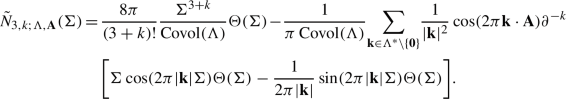(46)

The series is unconditionally summable in $${\mathcal {S}'(\mathbb {R}^{\ge 0})}$$, so $$\tilde{N}_{3,k;\Lambda ,\textbf{A}}$$ is a well-defined element of $$\mathcal {S}'(\mathbb {R}^{\ge 0})$$.

All of the computations in this section will be done in $$\mathcal {S}'(\mathbb {R}^{\ge 0})$$.

The function

\begin{aligned} \partial ^{-k} \Big [ \Sigma \cos (2\pi |\textbf{k}| \Sigma ) \Theta (\Sigma ) - (2\pi |\textbf{k}|)^{-1} \sin (2\pi |\textbf{k}| \Sigma ) \Theta (\Sigma )\Big ] \end{aligned}
(47)

is a k-fold iterated definite integral of $$\sigma \cos (2\pi |\textbf{k}| \sigma ) - (2\pi |\textbf{k}|)^{-1} \sin (2\pi |\textbf{k}| \sigma )$$ along $$\sigma \in [0,\Sigma ]$$.

Let $$\Sigma _{\textbf{k}} = 2\pi |\textbf{k}| \Sigma$$, so that $$\partial _\Sigma = 2\pi |\textbf{k}| \partial _{\Sigma _{\textbf{k}}}$$. Then, $$\partial _\Sigma ^{-k} = (2\pi )^{-k} |\textbf{k}|^{-k} \partial _{\Sigma _{\textbf{k}}}^{-k}$$, so

\begin{aligned} \partial ^{-k} \Bigg [ \Sigma \cos ( \Sigma _{\textbf{k}}) - \frac{1}{2\pi |\textbf{k}|} \sin (\Sigma _{\textbf{k}})\Bigg ] = (2\pi )^{-(k+1)} |\textbf{k}|^{-(k+1)} \partial ^{-k}_{\Sigma _{\textbf{k}}} [ \Sigma _{\textbf{k}} \cos (\Sigma _{\textbf{k}}) - \sin (\Sigma _{\textbf{k}})]. \end{aligned}
(48)

It can be seen inductively that there exist $$\alpha _k,\beta _k,\gamma _k,\delta _k \in \mathbb {R}$$ and polynomials $$Q_k\in \mathbb {R}[\Sigma ]$$ such that

\begin{aligned} \partial ^{-k}_{\Sigma } [ \Sigma \cos (\Sigma ) - \sin (\Sigma )] = Q_k(\Sigma ) + \alpha _k \Sigma \cos \Sigma + \beta _k \Sigma \sin \Sigma + \gamma _k \cos \Sigma + \delta _k \sin \Sigma . \end{aligned}
(49)

Plugging this into the computation above,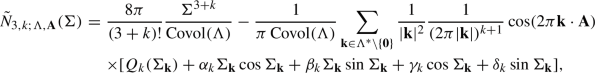(50)

where we are now omitting the factors of $$\Theta (\Sigma )$$, both sides being interpreted as elements of $$\mathcal {S}'(\mathbb {R}^{\ge 0})$$.

So, in order to compute $$\tilde{N}_{3,k;\Lambda ,\textbf{A}}$$ for $$k\ge 1$$, all that needs to be done is compute $$\alpha _k,\beta _k,\gamma _k,\delta _k$$, and $$Q_k$$.

Set $$\alpha _0 = 1, \beta _0 = 0, \gamma _0 = 0, \delta _0 = -1$$. Since $$\alpha _k$$, $$\beta _k$$, $$\gamma _k$$, $$\delta _k$$ can be defined in terms of $$\alpha _{k-1},\beta _{k-1},\gamma _{k-1},\delta _{k-1}$$ via a system of linear equations whose coefficients do not depend on k,

\begin{aligned} \begin{pmatrix} \alpha _k \\ \beta _k \\ \gamma _k \\ \delta _k \end{pmatrix} = \begin{pmatrix} m_{11} &{}\quad m_{12} &{}\quad m_{13} &{}\quad m_{14} \\ m_{21} &{}\quad m_{22} &{}\quad m_{23} &{}\quad m_{24} \\ m_{31} &{}\quad m_{32} &{}\quad m_{33} &{}\quad m_{34} \\ m_{41} &{}\quad m_{42} &{}\quad m_{43} &{}\quad m_{44} \\ \end{pmatrix}^k \begin{pmatrix} \alpha _0 \\ \beta _0 \\ \gamma _0 \\ \delta _0 \end{pmatrix} \end{aligned}
(51)

for some matrix $$M=\{m_{ij}\}_{i,j=1}^4$$ with entries $$m_{ij} \in \mathbb {R}$$. From

\begin{aligned} \int _0^\Sigma \sigma \cos (\sigma ) \,\textrm{d}\sigma = -1 + \cos (\Sigma ) + \Sigma \sin (\Sigma ), \quad \int _0^\Sigma \sigma \sin (\sigma ) \,\textrm{d}\sigma = - \Sigma \cos (\Sigma ) + \sin (\Sigma ), \end{aligned}
(52)

along with $$\int _0^\Sigma \cos (\sigma ) \,\textrm{d}\sigma = \sin (\Sigma )$$ and $$\int _0^\Sigma \sin (\sigma ) \,\textrm{d}\sigma = 1 - \cos (\Sigma )$$, we conclude that the only nonzero matrix entries are $$m_{12} = -1$$, $$m_{21} = +1$$, $$m_{31} = +1$$, $$m_{34} = -1$$, $$m_{42}=+1$$, and $$m_{43}=+1$$. That is,

\begin{aligned} M = \begin{pmatrix} 0 &{}\quad -1 &{}\quad 0 &{}\quad 0 \\ +1 &{}\quad 0 &{}\quad 0 &{}\quad 0 \\ +1 &{}\quad 0 &{}\quad 0 &{}\quad -1 \\ 0 &{}\quad +1 &{}\quad +1 &{}\quad 0 \\ \end{pmatrix} = \begin{pmatrix} J &{}\quad 0 \\ 1 &{}\quad J \end{pmatrix}, \end{aligned}
(53)

where $$J \in \mathbb {R}^{2\times 2}$$ is the matrix corresponding to a clockwise $$90^\circ$$ rotation of $$\mathbb {R}^2$$. We inductively deduce that, for all $$k\in \mathbb {N}$$,

\begin{aligned} M^k = \begin{pmatrix} J^{k} &{} 0 \\ kJ^{k-1} &{} J^{k} \end{pmatrix}. \end{aligned}
(54)

We have $$J^k = 1$$ if $$k=0 \bmod 4$$, $$J^k = J$$ if $$k=1 \bmod 4$$, $$J^k = -1$$ if $$k=2 \bmod 4$$, and $$J^k = -J$$ if $$k=3 \bmod 4$$; so, $$M^k$$ is given by

\begin{aligned} \begin{pmatrix} 0 &{} -1 &{} 0 &{} 0 \\ +1 &{} 0 &{} 0 &{} 0 \\ +k &{} 0 &{} 0 &{} -1 \\ 0 &{} +k &{} +1 &{} 0 \\ \end{pmatrix}, \; \begin{pmatrix} -1 &{} 0 &{} 0 &{} 0 \\ 0 &{} -1 &{} 0 &{} 0 \\ 0 &{} -k &{} -1 &{} 0 \\ +k &{} 0 &{} 0 &{} -1 \\ \end{pmatrix}, \; \begin{pmatrix} 0 &{} +1 &{} 0 &{} 0 \\ -1 &{} 0 &{} 0 &{} 0 \\ -k &{} 0 &{} 0 &{} +1 \\ 0 &{} -k &{} -1 &{} 0 \\ \end{pmatrix}, \; \begin{pmatrix} +1 &{} 0 &{} 0 &{} 0 \\ 0 &{} +1 &{} 0 &{} 0 \\ 0 &{} +k &{} +1 &{} 0 \\ -k &{} 0 &{} 0 &{} +1 \\ \end{pmatrix}\nonumber \\ \end{aligned}
(55)

in the four cases $$k=1,2,3,4\bmod 4$$ respectively.

Using these in Eq. (51), we have $$\alpha _k = \Re [i^k]$$, $$\beta _k = -\Im [i^k]$$, $$\gamma _k =(k+1) \Im [i^k]$$, $$\delta _k = - (k+1) \Re [i^k]$$. From the computations above, we also see that

\begin{aligned} Q_k(\Sigma ) = \int _0^\Sigma Q_{k-1}(\sigma )\,\textrm{d}\sigma - \alpha _{k-1} + \delta _{k-1} = \int _0^\Sigma Q_{k-1}(\sigma )\,\textrm{d}\sigma - (k+1)\Im [i^{k}].\nonumber \\ \end{aligned}
(56)

The jth component of this, $$[Q_k]_j$$, is given by $$(j!)^{-1} \partial ^j Q_k(0) = (j!)^{-1}Q_{k-j}(0) = - (k-j+1) \Im [i^{k-j}] / j!$$ if $$k-j \ge 1$$ and 0 otherwise. So,

\begin{aligned} Q_k(\Sigma ) = \sum _{j=0}^{k-1} (k-j+1) \Im [i^{k-j+2}]\frac{\Sigma ^j}{j!}. \end{aligned}
(57)

We therefore conclude that, for all $$k\in \mathbb {N}$$,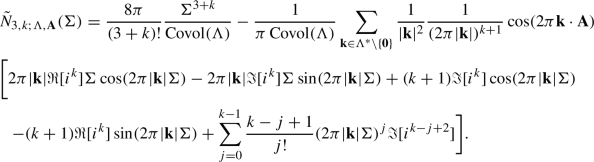(58)

We may pull out the polynomial part of the sum and write (adding back in the factors of $$\Theta$$ for later reference)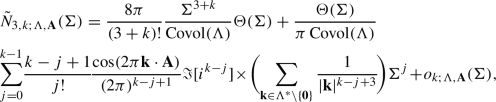(59)

for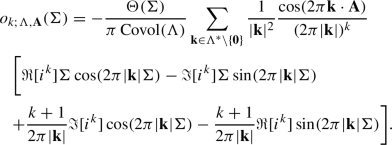(60)

By comparing $$o_{k;\Lambda ,\textbf{A}}$$ with a volume integral:

### Lemma 3.1

Fix $$k\ge 2$$. Given any compact $$K\subseteq [0,\infty )$$, the series on the right-hand side of Eq. (58) is uniformly convergent in K, so $${\tilde{N}_{3,k;\Lambda ,\textbf{A}}}$$, as defined by Eq. (59), can be considered as an element of $$C^0[0,\infty )$$.

Furthermore, $$\tilde{N}_{3,k;\Lambda ,\textbf{A}}(0) = 0$$ for all $$k\ge 2$$, so $$\tilde{N}_{3,k;\Lambda ,\textbf{A}}$$ can be considered as an element of $$C^0(\mathbb {R})$$. Similar statements apply to $$o_{k;\Lambda ,\textbf{A}}$$.

### Proposition 3.2

For each $$k\ge 0$$, the formal series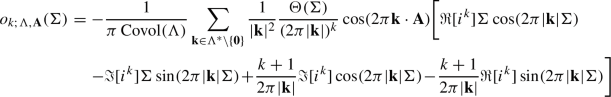(61)

is unconditionally summable in $$\mathcal {S}'(\mathbb {R})$$, hence Eq. (59) defines an element of $$\mathcal {S}'(\mathbb {R})$$.

### Proof

Given Lemma 3.1, the proposition holds for $$k\ge 2$$. We see that, for $$k\in \mathbb {N}^+$$,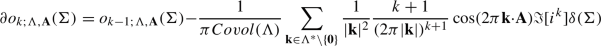(62)

at the level of formal series in $$\dot{\mathcal {S}}'(\mathbb {R}^{\ge 0})$$. For $$k=2$$, the second formal series in Eq. (62) is just zero, from which it follows that the series in Eq. (61) is unconditionally summable in $$\mathcal {S}'(\mathbb {R})$$ for $$k=1$$ and that the derivative of (61) (as an element of $$\mathcal {S}'(\mathbb {R})$$) is equal to the sum. For $$k=1$$, the second formal series in Eq. (62) is absolutely convergent, from which it follows that the series in Eq. (61) is unconditionally summable in $$\mathcal {S}'(\mathbb {R})$$ for $$k=0$$ and that the derivative of Eq. (61) as an element of $$\mathcal {S}'(\mathbb {R})$$ is equal to the sum. $$\square$$

The preceding proposition shows that Eq. (61) defines, for each $$k\in \mathbb {N}$$, a tempered distribution, and moreover that Eq. (62) holds in the usual distributional sense. Manifestly,

\begin{aligned} o_{k;\Lambda ,\textbf{A}}(\Sigma ), \tilde{N}_{3,k;\Lambda ,\textbf{A}}(\Sigma ) \in \dot{\mathcal {S}}'(\mathbb {R}^{\ge 0}) \end{aligned}
(63)

In other words, the given equation is an unconditionally convergent series in $$\dot{\mathcal {S}}'(\mathbb {R}^{\ge 0})$$.

Reversing the formal integration that led us to Eq. (59),

\begin{aligned} \partial \tilde{N}_{3,k;\Lambda ,\textbf{A}} = \tilde{N}_{3,k-1;\Lambda ,\textbf{A}} \end{aligned}
(64)

in $$\mathcal {S}'(\mathbb {R})$$ for $$k\in \mathbb {N}^+$$. Let $$\tilde{\Delta } = \partial \tilde{N}_{3,0;\Lambda ,\textbf{A}} \in \mathcal {S}'(\mathbb {R})$$. This is given by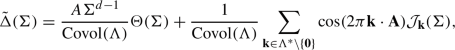(65)

where the sum is unconditionally summable in $$\mathcal {S}'(\mathbb {R})$$.

### Proposition 3.3

As an element of $$\mathcal {S}'(\mathbb {R})$$,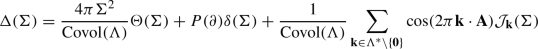(66)

for some polynomial $$P=P_{\Lambda ,\textbf{A}}$$ whose even order terms are all zero.

### Proof

Consider the support of $$E(\Sigma ) = \Delta (\Sigma ) - \tilde{\Delta }(\Sigma )$$.

• By Proposition 2.1, E is supported on $$(-\infty ,0]$$.

• Since both $$\Delta$$ and $$\tilde{\Delta }$$ are supported on $$[0,\infty )$$, the same is true for E.

So, E is supported at the origin. Since the only distributions supported on points are linear combinations of $$\delta$$-functions and derivatives thereof [22, Theorem 5.5], Eq. (66) holds for some polynomial P.

Given Schwartz $$F\in \mathcal {S}(\mathbb {R})$$,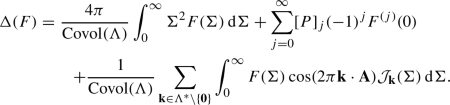(67)

Applying this for arbitrary even F and comparing with Eq. (28), which held for any even $$F \in \mathcal {S}(\mathbb {R})$$, we conclude that the even order terms of P all vanish. $$\square$$

Put differently, $$\Delta (\Sigma ) = P(\partial )\delta (\Sigma ) + \tilde{\Delta }(\Sigma )$$. Since integrals in $$\dot{\mathcal {S}}'(\mathbb {R}^{\ge 0})$$ are unique,

\begin{aligned} N_{3,k;\Lambda ,\textbf{A}}(\Sigma ) = \sum _{j=0}^k [P]_j \frac{\Sigma ^{k-j}}{(k-j)!} \Theta (\Sigma ) + \sum _{j=k+1}^\infty [P]_j \delta ^{(j-k-1)}(\Sigma ) + \tilde{N}_{3,k;\Lambda ,\textbf{A}}(\Sigma ) \end{aligned}
(68)

for each $$k\ge 1$$. By Lemma 3.1, $$\tilde{N}_{3,k;\Lambda ,\textbf{A}}(\Sigma )$$ is continuous for $$k\ge 2$$. Obviously, the same holds for $$N_{3,k;\Lambda ,\textbf{A}}$$ for $$k\ge 2$$, so Eq. (68) forces that $$[P]_2,[P]_3,[P]_4,\cdots$$ are all zero (though we already knew that $$[P]_2,[P]_4,\cdots$$ were all zero). By the previous proposition, $$[P]_0$$ as well, so

\begin{aligned} P(\partial ) = C \partial \end{aligned}
(69)

for some $$C\in \mathbb {C}$$.

### Proposition 3.4

The coefficient C in Eq. (69) is equal to 0.

### Proof

We examine Eq. (68) for $$k=1$$. Taking into account the vanishing of $$[P]_j$$ for $$j\ne 1$$, this says that $${N_{3,1;\Lambda ,\textbf{A}}(\Sigma ) = C \Theta (\Sigma ) + \tilde{N}_{3,1;\Lambda ,\textbf{A}}}$$. Since $$C \Theta : \mathbb {R}\rightarrow \mathbb {C}$$ is a piecewise continuous function and $$N_{3,1;\Lambda ,\textbf{A}}:\mathbb {R}\rightarrow \mathbb {R}$$ is a continuous function, $${\tilde{N}_{3,1;\Lambda ,\textbf{A}} = N_{3,1;\Lambda ,\textbf{A}} - C \Theta }$$ (considered as an element of $$\mathcal {S}'(\mathbb {R})$$) is a piecewise continuous function (meaning, of course, the tempered distribution corresponding via the standard correspondence to some piecewise continuous function, which we also call “$$\tilde{N}_{3,1;\Lambda ,\textbf{A}}$$”). Then, $$\tilde{N}_{3,1;\Lambda ,\textbf{A}}(\Sigma ) = N_{3,1;\Lambda ,\textbf{A}}(\Sigma ) - C \Theta (\Sigma )$$ holds in the ordinary sense for all $$\Sigma \ne 0$$. Consequently,

\begin{aligned} \lim _{\Sigma \rightarrow 0^+} \tilde{N}_{3,1;\Lambda ,\textbf{A}}(\Sigma ) = \lim _{\Sigma \rightarrow 0^+} N_{3,1;\Lambda ,\textbf{A}}(\Sigma ) - C = -C. \end{aligned}
(70)

Given any $$\chi \in C_{\textrm{c}}^\infty (\mathbb {R})$$, from the unconditional convergence, in $$\mathcal {S}'(\mathbb {R})$$, of the series defining $$\tilde{N}_{3,1;\Lambda ,\textbf{A}}$$,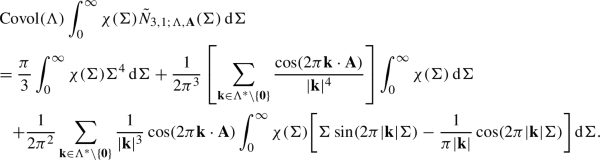(71)

Now suppose that $$\chi$$ is supported in $$(0,+\varepsilon )$$, $$0<\varepsilon <1$$. In the following computations, we use big-O notation with bounds independent of $$\chi ,\varepsilon$$, and parameters rR (introduced below). We bound each of the terms in Eq. (71):

• The first is $$\int _0^\infty \chi (\Sigma ) \Sigma ^4 \,\textrm{d}\Sigma = O(\varepsilon ^4 \Vert \chi \Vert _{L^1})$$.

• For any $$r>1$$,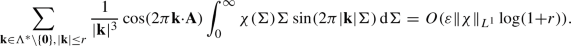(72)
• For any $$r>1$$,

\begin{aligned}{} & {} \sum _{\textbf{k}\in \Lambda ^*, |\textbf{k}|>r} \frac{1}{|\textbf{k}|^{3}} \cos (2\pi \textbf{k}\cdot \textbf{A})\int _0^\infty \chi (\Sigma ) \Sigma \sin (2\pi |\textbf{k}| \Sigma ) \,\textrm{d}\Sigma \nonumber \\{} & {} \quad = \sum _{|\textbf{k}|>r} \frac{1}{2\pi |\textbf{k}|^4} \cos (2\pi \textbf{k}\cdot \textbf{A}) \int _0^\infty [\chi (\Sigma ) + \Sigma \chi '(\Sigma )] \cos (2\pi |\textbf{k}| \Sigma ) \,\textrm{d}\Sigma \nonumber \\{} & {} \quad = O(r^{-1} (\Vert \chi \Vert _{L^1} + \varepsilon \Vert \chi ' \Vert _{L^1})). \end{aligned}
(73)
• Since $$|1-\cos (2\pi |\textbf{k}|\Sigma )| \le 2\pi ^2 |\textbf{k}|^2 \Sigma ^2$$ for all $$\Sigma \in \mathbb {R}$$,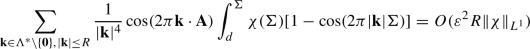for any $$R>1$$.

• On the other hand, just using a sup bound,

\begin{aligned} \sum _{\textbf{k}\in \Lambda ^*, |\textbf{k}|>R} \frac{1}{|\textbf{k}|^4} \cos (2\pi \textbf{k}\cdot \textbf{A}) \int _d^\Sigma \chi (\Sigma ) [1-\cos (2\pi |\textbf{k}| \Sigma )] = O(R^{-1} \Vert \chi \Vert _{L^1}). \end{aligned}

Combining these estimates, we conclude that

\begin{aligned} \int _0^\infty \chi (\Sigma ) \tilde{N}_{3,1;\Lambda ,\textbf{A}}(\Sigma ) \,\textrm{d}\Sigma = O((\varepsilon \log (1+r) +\varepsilon ^2 R+ R^{-1} + r^{-1})\Vert \chi \Vert _{L^1} + r^{-1} \varepsilon \Vert \chi ' \Vert _{L^1}). \end{aligned}
(74)

We now fix $$\chi _1 \in C_{\textrm{c}}^\infty (\mathbb {R})$$, supported in the open unit interval and satisfying $$\int _0^1 \chi _1(\sigma )\,\textrm{d}\sigma = 1$$, and we define rescalings $${\chi _\varepsilon = \varepsilon ^{-1} \chi _1(\varepsilon ^{-1} \sigma )}$$ for each $$\varepsilon \in (0,1)$$. Then $$\Vert \chi _\varepsilon \Vert _{L^1} = \Vert \chi _1 \Vert _{L^1}$$ and $$\Vert \chi _\varepsilon ' \Vert _{L^1} = \varepsilon ^{-1} \Vert \chi _1' \Vert _{L^1}$$. So, Eq. (74) says

\begin{aligned} \int _0^\infty \chi _{\varepsilon }(\Sigma ) \tilde{N}_{3,1;\Lambda ,\textbf{A}}(\Sigma ) \,\textrm{d}\Sigma = O(\varepsilon \log (1+r) + \varepsilon ^2 R + R^{-1} + r^{-1}). \end{aligned}
(75)

We can choose $$r=R = \varepsilon ^{-1}$$ to conclude that

\begin{aligned} \lim _{\varepsilon \rightarrow 0^+} \int _0^\infty \chi _{\varepsilon }(\Sigma ) \tilde{N}_{3,1;\Lambda ,\textbf{A}}(\Sigma ) \,\textrm{d}\Sigma = 0. \end{aligned}
(76)

By the right-continuity of $$\tilde{N}_{3,1;\Lambda ,\textbf{A}}$$ at the origin, the left-hand side of Eq. (76) is $$\lim _{\varepsilon \rightarrow 0^+} \tilde{N}_{3,1;\Lambda ,\textbf{A}}(\varepsilon )=-C$$. We can therefore conclude that $$C=0$$. $$\square$$

Plugging this information into Eq. (68), we can deduce the main proposition of this section:

### Proposition 3.5

For each $$k \in \mathbb {N}$$,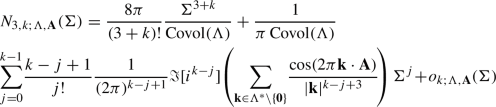(77)

as an element of $$\dot{\mathcal {S}}'(\mathbb {R}^{\ge 0})$$, where $$o_{k;\Lambda ,\textbf{A}} \in \dot{\mathcal {S}}'(\mathbb {R}^{\ge 0})$$ is given by Eq. (60).

Comparing Proposition 3.5 with Eqs. (18) and (19), we conclude Theorem 1.2.

## 4 The asymptotics of $$N_{3,k;\Lambda ,\textbf{A}}$$, $$k\ge 1$$, directly (sans sharpness)

For $$k\ge 2$$, there exist constants $$c_k$$ such that $$|o_{k;\Lambda ,\textbf{A}}(\Sigma )| \le c_k \Sigma$$ for all $$\Sigma \ge 0$$. This follows from the convergence of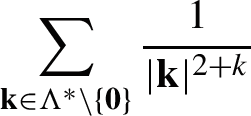(78)

for $$k\ge 2$$, which can be proven e.g. via comparison with a volume integral. We can therefore conclude that, for $$k\ge 2$$,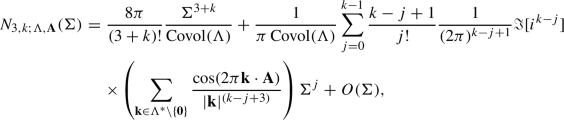(79)

as for all $$\Sigma > 0$$. Hence, Theorem 1.4 follows immediately from Theorem 1.2. The remainder of this section is devoted to the $$k=1$$ case.

For $$k=1$$, Eq. (78) is harmonically divergent, and so if it is the case that the series in Eq. (60) is convergent for given $$\Sigma >0$$, it is going to only be conditionally convergent. Nevertheless, we can still prove that

\begin{aligned} N_{3,1;\Lambda ,\textbf{A}}(\Sigma ) = \frac{\pi \Sigma ^4}{3{\text {Covol}}(\Lambda )} + O(\Sigma \log \Sigma ) \end{aligned}
(80)

as $$\Sigma \rightarrow \infty$$. By Eq. (59),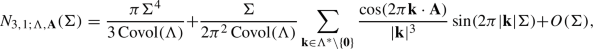(81)

in the sense that the left-hand side, viewed as an element of $$\mathcal {S}'(\mathbb {R}^{\ge 0})$$, and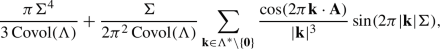(82)

also viewed as an element of $${\mathcal {S}'(\mathbb {R}^{\ge 0}_\Sigma )}$$, differ by an element of $${\mathcal {S}'(\mathbb {R}^{\ge 0})}$$ lying in $$\langle \Sigma \rangle L^\infty (\mathbb {R}^{\ge 0})$$. In fact, we can replace $$\langle \Sigma \rangle L^\infty (\mathbb {R}^{\ge 0})$$ with $$\langle \Sigma \rangle C_{\textrm{b}}^0(\mathbb {R}^{\ge 0})$$ in the previous sentence, where $$C_{\textrm{b}}^0\,(\mathbb {R}^{\ge 0})$$ is the set of continuous bounded functions on the closed half-line.

Let $$\chi \in C_{\textrm{c}}^\infty (\mathbb {R})$$ be a smooth, compactly supported, and real-valued function supported within the open unit interval (0, 1), satisfying

\begin{aligned} \int _0^1 \chi (\sigma ) \,\textrm{d}\sigma = 1 \quad \text { and } \quad \int _0^1 \chi (\sigma ) \sigma ^k \,\textrm{d}\sigma = 0 \end{aligned}
(83)

for all $$k=1,2,3,4$$. Such a function exists, and indeed $$\chi$$ can be constructed as a linear combination of 5 bump functions via a linear algebraic argument:

• Let $$\phi _1,\ldots ,\phi _5 \in C_{\textrm{c}}^\infty ((0,1))$$ and $$\mathfrak {M}$$ be the $$5\times 5$$ matrix whose (ij)th entry is $${\int _0^1 \phi _i(\sigma ) \sigma ^j \,\textrm{d}\sigma }$$. Then, $$\chi$$ can be taken as a linear combination of $$\phi _1,\ldots ,\phi _5$$ if $$\det \mathfrak {M}\ne 0$$. Fix $$\phi _0 \in C_{\textrm{c}}^\infty (\mathbb {R})$$ with $$\phi _0(0)=1$$ and total mass one, so that, setting

\begin{aligned}\phi _i(\sigma ) = w^{-1}\phi _0(w^{-1}(\sigma -a_i)) \end{aligned}

for $$i=1,2,3,4,5$$, $$\mathfrak {M}$$ can be made arbitrarily close in operator norm to the Vandermonde matrix $$\mathfrak {V}$$ on $$a_1,\ldots ,a_5$$ (and thus $$\det \mathfrak {M}$$ made arbitrarily close to $$\det \mathfrak {V}$$) by taking $$w\rightarrow 0^+$$. If $$a_1,\ldots ,a_5$$ are distinct, then $$\det \mathfrak {V}\ne 0$$. So, for sufficiently small w, $$\det \mathfrak {M}\ne 0$$ too.

For each $$\Sigma _0>1$$, let $$\chi _{\Sigma _0^2}(\sigma ) = \varsigma _{\Sigma _0^2} \chi ( \varsigma _{\Sigma _0^2} (\sigma -\Sigma _0))$$ for $$\varsigma _{\Sigma _0^2} = 1/((\Sigma _0^2+1)^{1/2}-\Sigma _0) = \langle \Sigma _0 \rangle (1+O(1/\langle \Sigma _0^2\rangle ))$$. Note that

\begin{aligned} \int _{-\infty }^{+\infty } \chi _{\Sigma _0^2}(\sigma ) \,\textrm{d}\sigma = \int _{\Sigma _0}^{\sqrt{\Sigma _0^2+1}} \chi _{\Sigma _0^2}(\sigma ) \,\textrm{d}\sigma = 1. \end{aligned}
(84)

Then,

\begin{aligned}{} & {} \int _{0}^{\infty } \chi _{\Sigma _0^2}(\Sigma ) \int _0^\Sigma N_{3;\Lambda ,\textbf{A}}(\sigma ) \,\textrm{d}\sigma \,\textrm{d}\Sigma = \int _0^{\Sigma _0} N_{3;\Lambda ,\textbf{A}}(\sigma ) \,\textrm{d}\sigma \nonumber \\{} & {} \quad + \int _0^\infty \chi _{\Sigma _0^2}(\Sigma ) \int _{\Sigma _0}^\Sigma N_{3;\Lambda ,\textbf{A}}(\sigma ) \,\textrm{d}\sigma \,\textrm{d}\Sigma , \end{aligned}
(85)

which is

\begin{aligned}{} & {} \int _0^{\Sigma _0} N_{3;\Lambda ,\textbf{A}}(\sigma ) \,\textrm{d}\sigma + N_{3;\Lambda ,\textbf{A}}(\Sigma _0)\int _0^\infty \chi _{\Sigma _0^2}(\Sigma ) (\Sigma - \Sigma _0) \,\textrm{d}\Sigma \nonumber \\{} & {} \quad +\int _0^\infty \chi _{\Sigma _0^2}(\Sigma ) \int _{\Sigma _0}^\Sigma (N_{3;\Lambda ,\textbf{A}}(\sigma )-N_{3;\Lambda ,\textbf{A}}(\Sigma _0)) \,\textrm{d}\sigma \,\textrm{d}\Sigma . \end{aligned}
(86)

Per Gauss, the last term is $$O(\Sigma _0)$$. So,

\begin{aligned}&\int _{0}^{\infty } \chi _{\Sigma _0^2}(\Sigma ) \int _0^\Sigma N_{3;\Lambda ,\textbf{A}}(\sigma ) \,\textrm{d}\sigma \,\textrm{d}\Sigma \nonumber \\&\quad = \int _0^{\Sigma _0} N_{3;\Lambda ,\textbf{A}}(\sigma ) \,\textrm{d}\sigma + N_{3;\Lambda ,\textbf{A}}(\Sigma _0)\int _0^\infty \chi _{\Sigma _0^2}(\Sigma ) (\Sigma - \Sigma _0) \,\textrm{d}\Sigma + O(\Sigma _0) \nonumber \\&\quad = \int _0^{\Sigma _0} N_{3;\Lambda ,\textbf{A}}(\sigma ) \,\textrm{d}\sigma + \frac{N_{3;\Lambda ,\textbf{A}}(\Sigma _0)}{\varsigma _{\Sigma _0^2}} \int _0^1 \chi (\sigma )\sigma \,\textrm{d}\sigma +O(\Sigma _0) \nonumber \\&\quad = \int _0^{\Sigma _0} N_{3;\Lambda ,\textbf{A}}(\sigma ) \,\textrm{d}\sigma + O(\Sigma _0). \end{aligned}
(87)

On the other hand,

\begin{aligned} \int _0^\infty \chi _{\Sigma _0^2}(\Sigma ) \Sigma ^4 \,\textrm{d}\Sigma = \int _{0}^{1} \chi (\sigma ) \left( \frac{\sigma }{\varsigma _{\Sigma _0^2}} - \Sigma _0 \right) ^4 \,\textrm{d}\sigma = \Sigma _0^4. \end{aligned}
(88)

Integrating both sides of Eq. (81) against $$\chi _{\Sigma _0^2}$$ therefore yields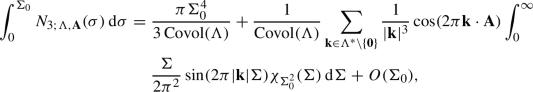(89)

where the infinite series is absolutely summable. We split the sum into two halves, one with $$|\textbf{k}| \le R$$ for to-be-determined $$R>0$$ and the (convergent) remainder. The first half is bounded as follows: noting that $$\Vert \chi _{\Sigma _0} \Vert _{L^1} =\Vert \chi \Vert _{L^1}$$,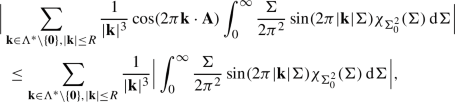(90)

which is bounded above by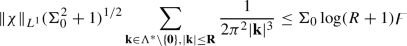(91)

for some $$\digamma =\digamma (\chi ,\Lambda )>0$$ that does not depend on $$\Sigma _0,R$$. The second half is bounded as follows:

\begin{aligned}{} & {} \Big |\sum _{\textbf{k}\in \Lambda ^*, |\textbf{k}|>R} \frac{1}{|\textbf{k}|^{3}} \cos (2\pi \textbf{k}\cdot \textbf{A}) \int _0^{\infty } \frac{\Sigma }{2\pi ^2} \sin (2\pi |\textbf{k}| \Sigma ) \chi _{\Sigma _0^2}(\Sigma ) \,\textrm{d}\Sigma \Big | \nonumber \\{} & {} \quad \le \Big |\sum _{\textbf{k}\in \Lambda ^*,|\textbf{k}|>R} \frac{1}{|\textbf{k}|^{4}} \cos (2\pi \textbf{k}\cdot \textbf{A}) \int _0^{\infty } \frac{\Sigma }{4\pi ^3} \cos (2\pi |\textbf{k}| \Sigma ) \chi _{\Sigma _0^2}'(\Sigma ) \,\textrm{d}\Sigma \Big | \nonumber \\{} & {} \qquad +\Big |\sum _{\textbf{k}\in \Lambda ^*,|\textbf{k}|>R}^\infty \frac{1}{|\textbf{k}|^{4}} \cos (2\pi \textbf{k}\cdot \textbf{A}) \int _0^{\infty } \frac{1}{4\pi ^3} \cos (2\pi |\textbf{k}| \Sigma ) \chi _{\Sigma _0^2}(\Sigma ) \,\textrm{d}\Sigma \Big |. \end{aligned}
(92)

Using $$\chi _{\Sigma _0^2}'(\Sigma ) = \varsigma _{\Sigma _0^2}^2 \chi '(\varsigma _{\Sigma _0^2}(\Sigma -\Sigma _0))$$,

\begin{aligned}{} & {} \Big |\sum _{\textbf{k}\in \Lambda ^*,|\textbf{k}|>R}^\infty \frac{1}{|\textbf{k}|^{4}} \cos (2\pi \textbf{k}\cdot \textbf{A}) \int _0^{\infty } \frac{\Sigma }{4\pi ^3} \cos (2\pi |\textbf{k}| \Sigma ) \chi _{\Sigma _0^2}'(\Sigma ) \,\textrm{d}\Sigma \Big | \nonumber \\{} & {} \quad = \varsigma _{\Sigma _0^2}^2 \Big |\sum _{\textbf{k}\in \Lambda ^*,|\textbf{k}|>R}^\infty \frac{1}{|\textbf{k}|^{4}} \cos (2\pi \textbf{k}\cdot \textbf{A}) \int _0^{\infty } \frac{\Sigma }{4\pi ^3} \cos (2\pi |\textbf{k}| \Sigma ) \chi '(\varsigma _{\Sigma _0^2}(\Sigma -\Sigma _0 )) \,\textrm{d}\Sigma \Big |\nonumber \\ \end{aligned}
(93)

is bounded above by

\begin{aligned} \digamma \Sigma _0^2 \sum _{\textbf{k}\in \Lambda ^*, |\textbf{k}|>R}^\infty \frac{1}{|\textbf{k}|^{4}}= O(\Sigma _0^2 R^{-1}) \end{aligned}
(94)

for some $$\digamma >0$$ (not necessarily the same as before), and likewise for the second term on the right-hand side of Eq. (92). So, if $$R>1$$,

\begin{aligned} \int _0^{\Sigma _0} N_{3;\Lambda ,\textbf{A}}(\sigma ) \,\textrm{d}\sigma = \frac{\pi \Sigma _0^4}{3 {\text {Covol}}(\Lambda )} + O(\Sigma _0 \log R ) + O(\Sigma _0^2 R^{-1}). \end{aligned}
(95)

We can therefore take $$R = \Sigma _0$$ and conclude that

\begin{aligned} \int _0^\Sigma N_{3;\Lambda ,\textbf{A}}(\sigma ) \,\textrm{d}\sigma = \frac{\pi }{3} \frac{\Sigma ^4}{{\text {Covol}}(\Lambda )} + O(\Sigma \log \Sigma ) \end{aligned}
(96)

as $$\Sigma \rightarrow \infty$$. We have therefore proven Theorem 1.3.

## 5 The asymptotics of $$N_{3,k;\Lambda ,\textbf{A}}$$, $$k\ge 2$$, via the Fourier transform

Missing from the previous section is a proof that the asymptotic expansions in Eqs. (79) and (80) are the best asymptotic expansions possible (assuming, at least, that we are only allowed to use polynomials). In this section we will give another proof of these expansions, one which makes it easy to deduce sharpness. While somewhat more involved than the direct arguments above, it uses only structural features of $$\mathcal {F}N_{3,0;\Lambda ,\textbf{A}}$$ which are guaranteed to hold on conceptual grounds – the relation to the wave equation on the standard 3-torus – so this approach has some appeal. Note that while we compute out $$\mathcal {F}N_{3,0;\Lambda ,\textbf{A}}$$ fairly explicitly, we only use this computation in order to prove the estimate Proposition 5.6 quickly. For the sake of Corollary 1.3, Corollary 1.4, Theorem 1.5, what matters is the truth of this estimate, not the method of proof. We proceed via an $$L^1,L^2$$-based analysis of the Fourier transform $$\mathcal {F}N_{3;\Lambda ,\textbf{A}}=\mathcal {F}N_{3;\Lambda ,\textbf{A}}(\tau )$$ – defined using the conventions specified in the introduction, Eq. (14) – whose singularity structure we analyze in detail. The results below also serve to verify the hypotheses of the Tauberian theorem in , which, as stated there (without proof), applies to the 3-torus.

It is straightforward to compute that:

### Proposition 5.1

For each $$r\ge 0$$, the Fourier transform $$\mathcal {F}J\in \mathcal {S}'(\mathbb {R})$$ of the function $$J(\Sigma )=\Theta (\Sigma ) \Sigma ^{3/2} J_{1/2}(2\pi r \Sigma )$$ is

\begin{aligned} \mathcal {F}J(\tau ) = 4 i r^{1/2}\frac{ \tau }{( (\tau -i0)^2-4 \pi ^2 r^2)^2 }= 4 i r^{1/2} \frac{ \tau -i0}{( (\tau -i0)^2-4 \pi ^2 r^2)^2 }, \end{aligned}
(97)

i.e. the limit $$\lim _{\epsilon \rightarrow 0^+} H_\epsilon \in \mathcal {S}'(\mathbb {R})$$ (in the topology of $$\mathcal {S}'(\mathbb {R})$$) of $$H_\epsilon (\tau ) = 4 i r^{1/2} (\tau -i \epsilon ) ((\tau - i \epsilon )^2-4\pi ^2 r^2)^{-2}$$.

Let $$j\in \mathbb {R}^+$$. (For the present paper, it suffices to consider $$j\in \mathbb {N}^+$$, and for applications to Weyl’s law more generally, $$j\in \mathbb {N}^+/2$$ should suffice.) For each $$s\in \mathbb {R}$$, we have a well-defined Fourier multiplier $$\langle D \rangle ^{-s}: \mathcal {S}'(\mathbb {R})\rightarrow \mathcal {S}'(\mathbb {R})$$. For each $$p\in [1,\infty )$$, we let $$\mathcal {S}'(\mathbb {R})\cap L^p_{\textrm{loc}}(\mathbb {R})$$ denote the set of tempered distributions locally in $$L^p(\mathbb {R})$$.

### Proposition 5.2

Let $$j\ge 1$$. For each $$T,s \in \mathbb {R}$$ and $$p\in [1,\infty )$$, $$(\tau -T \pm i0)^{-j} \in \langle D \rangle ^{-s}( \mathcal {S}'(\mathbb {R})\cap L^p_{\textrm{loc}}(\mathbb {R}))$$ if and only if $$s< p^{-1} - j$$.

### Proof

By the translation invariance of $$\langle D \rangle ^{-s}$$, it suffices to consider the case $$T=0$$, that is to show that

\begin{aligned} \langle D \rangle ^s \frac{1}{(\tau \pm i0)^j} \in L^p_{\textrm{loc}}(\mathbb {R}) \end{aligned}
(98)

under and only under the stated conditions. Via complex conjugation, it suffices to consider the case of $$P_{s,j}(\tau ) = \langle D \rangle ^s (\tau -i0)^{-j}$$. Claim: if $$j+s$$ is not an integer, then there exists (I) some sj-dependent sequence $$\{c_\ell \}_{\ell =0}^\infty$$ containing only finitely many nonzero entries and (II) some $$E \in C^0(\mathbb {R})$$ such that

\begin{aligned} P_{s,j}(\tau ) = \sum _{\ell =0}^\infty \frac{c_\ell }{(\tau - i0)^{j+s - \ell }} + E(\tau ), \end{aligned}
(99)

where $$c_0 \ne 0$$. Indeed, $$\mathcal {F}^{-1} P_{s,j}(\sigma ) = \langle \sigma \rangle ^s \mathcal {F}^{-1} P_{0,j}(\sigma ) = \alpha _j\langle \sigma \rangle ^s \Theta (\sigma ) \sigma ^{j-1}$$ for some $$\alpha _j \in \mathbb {C}$$ [30, pg. 360]. For any $$S \in \mathbb {N}$$, for sufficiently large $$\sigma >0$$ we have

\begin{aligned} \langle \sigma \rangle ^s = E_S(\sigma ) + \sum _{n=0}^S \beta _{n,s} \sigma ^{s-n} \end{aligned}
(100)

for some $$E_S \in \langle \sigma \rangle ^{s-S-1} L^\infty [\sigma _0,\infty )$$, for some $$\sigma _0>0$$. Then,

\begin{aligned} \mathcal {F}^{-1} P_{s,j}(\sigma ) = \alpha _j \Theta (\sigma ) \sigma ^{j-1} E_S(\sigma ) + \sum _{n=0}^S \alpha _j \beta _{n,s} \Theta (\sigma ) \sigma ^{s+j-n-1} \end{aligned}
(101)

for $$\sigma >\sigma _0$$. Defining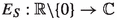by Eq. (101) for all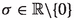, $$E_S \in \langle \sigma \rangle ^{s-S-1} L^\infty (\mathbb {R}) + \sigma ^{1-j} L^1_{\textrm{c}}(\mathbb {R})$$. If $$S<s+j$$ then Eq. (101) holds as an equality between tempered distributions. (For such S, $$\Theta (\sigma ) \sigma ^{s+j-n} \in L^1_{\textrm{loc}}(\mathbb {R}_\sigma )$$ for all $$n=0,\ldots ,S$$, so the right-hand side of Eq. (101) actually makes sense as a tempered distribution.) We take $$S = \lfloor s+j \rfloor$$, which is strictly less than $$s+j$$ if $$s+j$$ is not an integer. We have $$\sigma ^{j-1} \langle \sigma \rangle ^{s-S-1} L^\infty (\mathbb {R}) + \sigma ^{j-1} L^1_{\textrm{c}}(\mathbb {R}) \subset L^1(\mathbb {R})$$, so $$\Theta (\sigma ) \sigma ^{j-1} E_s(\sigma ) \in L^1(\mathbb {R})$$. Taking the Fourier transform, Eq. (99) holds for

\begin{aligned} E = \alpha _j \mathcal {F}[\Theta (\sigma )\sigma ^{j-1} E_S(\sigma )] \in C^0(\mathbb {R}), \end{aligned}
(102)

with $$c_\ell = 0$$ for $$\ell >j+s$$. (And we see that the leading order coefficient in the main sum, $$c_0$$, is nonzero.)

From Eq. (99), we conclude that $$P_{s,j} \in \mathcal {S}'(\mathbb {R})\cap L^p_{\textrm{loc}}(\mathbb {R})$$ if and only if $$j+s \le 0$$ or if $$\tau ^{-j-s} \in L^p_{\textrm{loc}}(\mathbb {R})$$, meaning that

\begin{aligned} \int _0^1 \frac{1}{\tau ^{pj+ps}} \,\textrm{d}\tau < \infty . \end{aligned}
(103)

This holds if and only if $$pj+ps < 1$$. (If $$j+s \le 0$$, this holds.) This is equivalent to $$s < p^{-1} - j$$. This completes the proof of the proposition, except when $$s+j$$ is an integer, which we handle by reduction to the generic case:

• If $$s<p^{-1} - j$$, then we can find some $$s_0$$ with $$s<s_0 < p^{-1} -j$$ and $$s_0 + j$$ is not an integer. The argument above therefore shows that $$P_{s_0,j} \in \mathcal {S}'(\mathbb {R})\cap L^p_{\textrm{loc}}(\mathbb {R})$$. We have $$P_{s,j} = \langle D \rangle ^{s - s_0} P_{s_0,j}$$. Since $$\langle D \rangle ^{s-s_0}: \mathcal {S}'(\mathbb {R})\rightarrow \mathcal {S}'(\mathbb {R})$$ is a Fourier multiplier (and a Kohn-Nirenberg $$\Psi$$DO) of negative order, the subspace $$\mathcal {S}'(\mathbb {R})\cap L^p_{\textrm{loc}}(\mathbb {R}) \subset \mathcal {S}'(\mathbb {R})$$ is closed under it, so $$P_{s,j} \in \mathcal {S}'(\mathbb {R})\cap L^p_{\textrm{loc}}(\mathbb {R})$$.

• If $$s> p^{-1} - j$$, then we can find some $$s_0$$ with $$s>s_0 > p^{-1} - j$$ and $$s_0 + j$$ is not an integer. If $$P_{s,j}$$ were in $$\mathcal {S}'(\mathbb {R})\cap L^p_{\textrm{loc}}(\mathbb {R})$$, then $$P_{s_0,j} = \langle D \rangle ^{-(s-s_0)} P_{s,j}$$ would be too. But the argument above shows that $$P_{s_0,j} \notin L^p_{\textrm{loc}}(\mathbb {R})$$.

• The one remaining case of the proposition is $$s = p^{-1} - j$$ and $$s+j \in \mathbb {Z}$$. Since $$p\in [1,\infty )$$, this can only hold if $$p=1$$. If $$P_{s,j}$$ were in $$\mathcal {S}'(\mathbb {R})\cap L^1_{\textrm{loc}}(\mathbb {R})$$, then, for any Schwartz $$\chi$$, $$(\mathcal {F}^{-1} \chi )P_{s,j} \in L^1(\mathbb {R})$$, which implies that

\begin{aligned} \chi (\sigma ) * \langle \sigma \rangle ^s \Theta (\sigma ) \sigma ^{j-1} \in C^0(\mathbb {R}) \end{aligned}
(104)

and satisfies

\begin{aligned} \lim _{\sigma \rightarrow \infty } \chi (\sigma ) * \langle \sigma \rangle ^s \Theta (\sigma ) \sigma ^{j-1} = 0. \end{aligned}
(105)

But, if $$\int _{-\infty }^{+\infty } \chi (\sigma )\,\textrm{d}\sigma \ne 0$$ then this is false, as $$\lim _{\sigma \rightarrow \infty } \chi (\sigma ) * \langle \sigma \rangle ^s \Theta (\sigma ) \sigma ^{j-1} = \int _{-\infty }^{+\infty }\chi (\sigma ) \,\textrm{d}\sigma$$.

$$\square$$

We deduce:

### Proposition 5.3

Suppose that $$f \in \mathcal {S}'(\mathbb {R})$$ is a tempered distribution with singular support at a single point $$T\in \mathbb {R}$$, and suppose that

\begin{aligned} f(\tau ) = \sum _{ j \in \mathcal {J}} \frac{\alpha _j}{(\tau -T\pm i0)^j} \bmod C^\infty \end{aligned}
(106)

in some neighborhood of T, for some finite subset $$\mathcal {J}\subset [1,\infty )$$ and some $$\alpha _j \in \mathbb {C}$$. Then, for $$s\in \mathbb {R}$$ and $$p\in [1,\infty )$$, $$f\in \langle D \rangle ^{-s} L^p_{\textrm{loc}}(\mathbb {R})$$ if and only if $${\text {max}} \mathcal {J}<p^{-1} - s$$.

### Proposition 5.4

Equation (16) holds, and the Fourier transform $$\mathcal {F}N_{3;\Lambda ,\textbf{A}}(\tau ) \in \mathcal {S}'(\mathbb {R}_\tau )$$ of $$N_{3;\Lambda ,\textbf{A}}$$ is given by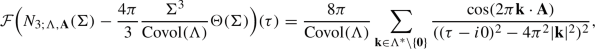(107)

where the sum on the right-hand side is (unconditionally) convergent in $$\mathcal {S}'(\mathbb {R}_\tau )$$.

### Proof

Since $$\mathcal {F}: \mathcal {S}'(\mathbb {R})\rightarrow \mathcal {S}'(\mathbb {R})$$ is continuous, the Fourier transform of the sum on the right-hand side of Proposition 3.3 is, up to a factor of $$1/{\text {Covol}}(\Lambda )$$,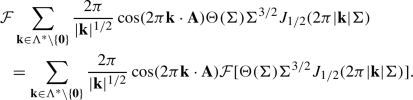(108)

By Proposition 5.1, the right-hand side is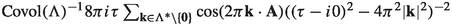.

Combining this with standard formula for $$\mathcal {F}[\Theta (\Sigma ) \Sigma ^2]$$ , we get Eq. (16). On the other hand, integrating, we get Eqs. (107), (17). $$\square$$

Consider now the case $$\Lambda =\mathbb {Z}^3,\textbf{A}=0$$. It is clear that the series,

\begin{aligned} \sum _{n=1}^\infty \frac{r_3(n)}{((\tau -i0)^2 - 4\pi ^2 n)^2}, \end{aligned}
(109)

where $$r_3(n)=\{(n_1,n_2,n_3)\in \mathbb {Z}^3:n_1^2+n_2^2+n_3^2=n\}$$, on the right-hand side of Eq. (107) is convergent in the topology of tempered distributions. It is not difficult to see that the series converges pointwise for all $${\tau \notin 2\pi \sqrt{\mathbb {N}^+}}$$. In fact, it converges uniformly on compact neighborhoods disjoint from $${2\pi \sqrt{\mathbb {N}^+}}$$ (and in fact the same holds for derivatives). At $$\tau \in {2\pi \sqrt{\mathbb {N}^+}}$$, only one term in Eq. (109) is singular, and the sum of the rest is uniformly convergent in some neighborhood. Consequently, the singularity of the right-hand side of Eq. (109) at $$\tau = 2 \pi \sqrt{n}$$ is no worse than the singularity of the term $$((\tau -i0)^2 - 4\pi ^2 n)^{-2}$$ at $$\tau = 2 \pi \sqrt{n}$$, and – assuming that $$r_3(n) \ne 0$$ – it is precisely as bad. So, we expect the local Sobolev regularity of $$\mathcal {F}(N_3(\Sigma ) - (4/3)\pi \Sigma ^3 \Theta (\Sigma ))(\tau )$$ to be explicitly analyzable by expanding $$((\tau -i0)^2 - 4\pi ^2 n)^{-2}$$ in Laurent series around $$\tau = 2 \pi \sqrt{n}$$. (The global regularity will require an additional argument.)

When computing the Fourier transforms of $$N_{3,k;\Lambda ,\textbf{A}}$$ for $$k\ge 1$$, we multiply by a nonzero power of $$1/\tau$$. For $$\tau \ne 0$$, the upshot of the previous paragraph remains unchanged, but this division produces a singularity at $$\tau = 0$$ in each term in Eq. (109). Let $$\tau _2 = \tau ^2$$. For $$r>0$$,

\begin{aligned} \frac{\partial ^k}{\partial \tau _2^k}\frac{1}{(\tau _2 - 4\pi ^2 r^2)^2} \Big |_{\tau _2 = 0} = \frac{ (k+1)!}{ (4\pi ^2 r^2)^{k+2}} = \frac{(k+1)!}{(2\pi )^{2k+4}} \frac{1}{r^{2k+4}}. \end{aligned}
(110)

The Kth order Taylor series of $$(\tau _2-4\pi ^2 r^2)^{-2}$$ in $$\tau _2$$ around $$\tau _2 = 0$$ is therefore

\begin{aligned} \sum _{k=0}^K \frac{\tau _2^k}{k!} \frac{\partial ^k}{\partial \tau _2^k}\frac{1}{(\tau _2 - 4\pi ^2 r^2)^2} \Big |_{\tau _2 = 0} = \sum _{k=0}^K \frac{k+1}{(2\pi )^{2k+4}} \frac{\tau _2^k}{r^{2k+4}}, \end{aligned}
(111)

which we consider as an even polynomial of $$\tau$$.

Consider, for each $$L\in \mathbb {N}$$, $$K\in \mathbb {N}\cup \{-1\}$$, the tempered distribution

\begin{aligned} F_{L,K,r}(\tau ) = \Bigg [\frac{1}{(\tau -i0)^{L}}\frac{1}{((\tau -i0)^2 - 4\pi ^2 r^2)^2} - \sum _{k=0}^K \frac{k+1}{(2\pi )^{2k+4}} \frac{1}{r^{2k+4}} \frac{1}{(\tau -i0)^{L-2k}} \Bigg ], \nonumber \\ \end{aligned}
(112)

which we will estimate.

• Via Taylor’s theorem, $$F_{L,K,r}$$ is a function for $$\tau$$ in some punctured neighborhood of the origin, of size $$O(\tau ^{2K+2-L})$$ as $$\tau \rightarrow 0$$. We will choose K so that the singularity of $$F_{L,K,r}(\tau )$$ at $$\tau = 0$$ is no greater than the singularity of $$(\tau _2 - 4\pi ^2 r^2)^{-2}$$ at $$\tau = \pm 2\pi r^2$$, that is second order. In other words, we should take $$2K+2-L \ge -2$$, i.e. $$K\ge \lceil L/2 \rceil - 2$$.

• As $$\tau \rightarrow \pm \infty$$, on the other hand, $$F_{L,K,r}(\tau ) = O(|\tau |^{2K-L})$$. In order for $$\langle \tau \rangle ^{2K-L}$$ to be integrable, we should have $$2K-L < -1$$, i.e. $$K<(L-1)/2$$.

In particular, $$K = \lceil L/2 \rceil - 2$$ satisfies our requirements.

### Proposition 5.5

For any $$L\in \mathbb {N}$$, $$\Vert \mathcal {F}F_{L,K,1} \Vert _{\langle \Sigma \rangle L^\infty (\mathbb {R}_\Sigma )} < \infty$$ if $$K= \lceil L/2 \rceil - 2$$.

### Proof

We choose $$\chi _1,\chi _2 \in C_{\textrm{c}}^\infty (\mathbb {R})$$ and $$\chi _3 \in \mathcal {S}(\mathbb {R})$$ such that $$\chi _1$$ is supported in a neighborhood of the origin away from $$2\pi$$, $$\chi _2$$ is supported in a neighborhood of $$2\pi$$ away from the origin, $$\chi _3$$ is identically zero in $$[-1,8]$$, and $$\chi _1+\chi _2+\chi _3 = 1$$. (Such functions exist.) Then

\begin{aligned} \Vert \mathcal {F}F_{L,K,1} \Vert _{\langle \Sigma \rangle L^\infty (\mathbb {R}_\Sigma )} \le \sum _{j=1}^3 \Vert \mathcal {F}\chi _j F_{L,K,1} \Vert _{\langle \Sigma \rangle L^\infty (\mathbb {R}_\Sigma )} . \end{aligned}
(113)

The function $$\chi _3 F_{L,K,1}$$ is smooth and absolutely integrable, so

\begin{aligned} \Vert \mathcal {F}\chi _3 F_{L,K,1} \Vert _{\langle \Sigma \rangle L^\infty (\mathbb {R}_\Sigma )}<\infty . \end{aligned}
(114)

On the other hand, $$\chi _1 F_{L,K,1} = E(\tau ) + \alpha \chi _1(\tau ) (\tau -i0)^{-2} + \beta \chi _1(\tau ) (\tau - i0)^{-1}$$ for some smooth, absolutely integrable E and some $$\alpha ,\beta \in \mathbb {C}$$. Then $$\mathcal {F}E$$ is continuous and uniformly bounded, and $$\mathcal {F}(\chi _1 (\tau -i0)^{-2})(\Sigma ) = \mathcal {F}\chi _1 * \mathcal {F}((\tau -i0)^{-2})(\Sigma )$$ is the convolution of a Schwartz function with an element of $$\langle \Sigma \rangle L^\infty (\mathbb {R}_\Sigma )$$ and is therefore in $$\langle \Sigma \rangle L^\infty (\mathbb {R}_\Sigma )$$ as well. An analogous argument applies to $$\mathcal {F}(\chi _1(\tau ) (\tau -i0)^{-1})(\Sigma )$$. We conclude that

\begin{aligned} \Vert \mathcal {F}\chi _1 F_{L,K,1} \Vert _{\langle \Sigma \rangle L^\infty (\mathbb {R}_\Sigma )}<\infty . \end{aligned}
(115)

A similar argument applies to the second term in Eq. (113), so

\begin{aligned} \Vert \mathcal {F}\chi _2 F_{L,K,1} \Vert _{\langle \Sigma \rangle L^\infty (\mathbb {R}_\Sigma )}<\infty . \end{aligned}
(116)

Combining the estimates Eqs. (114), (115), and (116) with Eq. (113), we get the result $$\Vert \mathcal {F}F_{L,K,1} \Vert _{\langle \Sigma \rangle L^\infty (\mathbb {R}_\Sigma )} < \infty$$. $$\square$$

### Proposition 5.6

If $$L\in \{2,3,4,\cdots \}$$ and $$K= \lceil L/2 \rceil -2$$,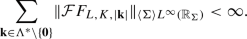(117)

### Proof

First note that $$F_{L,K,|\textbf{k}|}(\tau ) = |\textbf{k}|^{-4-L} F_{L,K,1}(\tau /|\textbf{k}|)$$. (Indeed, we can replace the $$\tau - i0$$ in Eq. (112) with $$|\textbf{k}|(\tau /|\textbf{k}|-i0)$$ and then factor out the factor of $$|\textbf{k}|$$.) Second, note that $$\mathcal {F}[F_{L,K,1}(\tau /|\textbf{k}|)](\sigma ) = |\textbf{k}| \mathcal {F}F_{L,K,1}(|\textbf{k}| \sigma )$$. Third,

\begin{aligned} \Vert \mathcal {F}F_{L,K,1}(|\textbf{k}|\sigma ) \Vert _{\langle \sigma \rangle L^\infty (\mathbb {R}_\sigma )}&= \Vert \langle \sigma \rangle ^{-1} \mathcal {F}F_{L,K,1}(|\textbf{k}| \sigma ) \Vert _{L^\infty (\mathbb {R}_\sigma )} \end{aligned}
(118)
\begin{aligned}&= \Vert \langle |\textbf{k}|^{-1} \sigma \rangle ^{-1} \mathcal {F}F_{L,K,1}( \sigma ) \Vert _{L^\infty (\mathbb {R}_\sigma )}. \end{aligned}
(119)

Fourth, $$\langle |\textbf{k}|^{-1} \sigma \rangle ^{-1} \le |\textbf{k}| \langle \sigma \rangle ^{-1}$$. Therefore, $$\Vert \mathcal {F}F_{L,K,|\textbf{k}|} \Vert _{\langle \sigma \rangle L^\infty (\mathbb {R}_\sigma )} \le |\textbf{k}|^{-2-L} \Vert \mathcal {F}F_{L,K,1} \Vert _{\langle \sigma \rangle L^\infty (\mathbb {R}_\sigma )}$$. It follows that(120)

This will be finite if (and only if) $$L>1$$ (and since L is an integer, $$L\ge 2$$) and $$\Vert \mathcal {F}F_{L,K,1} \Vert _{\langle \sigma \rangle L^\infty (\mathbb {R}_\sigma )} <\infty$$. By Proposition 5.5, this holds for the given K. $$\square$$

Since

\begin{aligned} N_{3,k;\Lambda ,\textbf{A}}'(\sigma ) = N_{3,k-1;\Lambda ,\textbf{A}}(\sigma ) \end{aligned}
(121)

holds as an identity of tempered distributions,

\begin{aligned} \mathcal {F}N_{3,k-1;\Lambda ,\textbf{A}}(\tau ) = \mathcal {F}[N_{3,k;\Lambda ,\textbf{A}}'](\tau ) = i \tau \mathcal {F}N_{3,k;\Lambda ,\textbf{A}}(\tau ). \end{aligned}
(122)

We inductively conclude (using Proposition A.4 repeatedly) that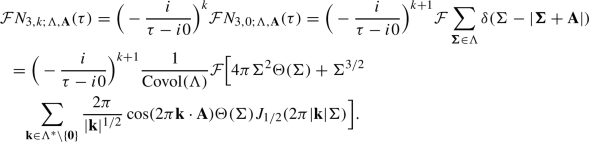(123)

Consequently, letting $$\bar{N}_{3,k;\Lambda ,\textbf{A}}(\Sigma ) = N_{3,k;\Lambda ,\textbf{A}}(\Sigma )- 8\pi {\text {Covol}}(\Lambda )^{-1} \Sigma ^{3+k} \Theta (\Sigma )/(3+k)!$$, we have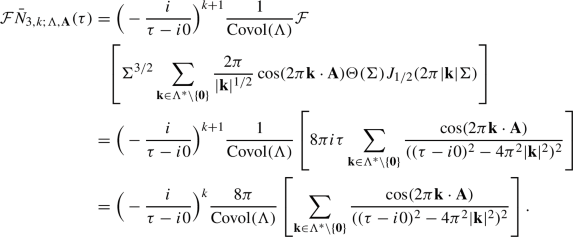(124)

For any $$K\in \mathbb {N}$$, we can rewrite Eq. (124) as follows (replacing the dummy variable ‘k’ with $$m \in \mathbb {N}$$ to avoid conflict):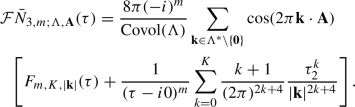(125)

Rearranging,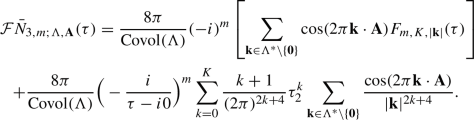(126)

(Note that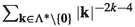is absolutely convergent for each $$k\ge 0$$.)

We now take $$m\ge 2$$ and $$K = \lceil m/2 \rceil - 2$$, so that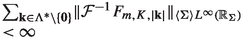, per the conclusion of Proposition 5.6.

Recall the following computation — cf. [30, pg. 360]: for each $$\ell \in \mathbb {R}^+$$,

\begin{aligned} \mathcal {F}^{-1}[(\tau -i0)^{-\ell }](\sigma ) = (+i)^{\ell } \Theta (\sigma ) \sigma ^{\ell -1} / (\ell -1)!. \end{aligned}
(127)

Applying $$\mathcal {F}^{-1}$$ to Eq. (126) and using the fact that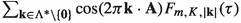is (unconditionally) convergent in $$\mathcal {S}'(\mathbb {R}_\tau )$$,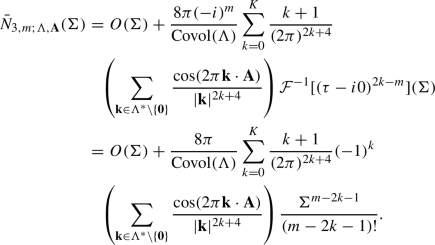(128)

We conclude – independently of §3,4 – that Eq. (22) holds, with $$C_{j;\Lambda ,\textbf{A}} = 0$$ if $$j \in \mathbb {N}$$ is odd and with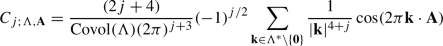(129)

if j is even.

### Proposition 5.7

For all $$k\in \mathbb {N}$$, there does not exist an $$\epsilon >0$$ such that, for some polynomial $$Z(\sigma ) = Z_{\Lambda ,\textbf{A}}(\sigma ) \in \mathbb {C}[\sigma ]$$, we have $$N_{3,k;\Lambda ,\textbf{A}}(\Sigma ) - Z(\Sigma ) = O(\Sigma ^{1-\epsilon })$$ as $$\Sigma \rightarrow \infty$$.

### Proof

If $$N_{3,k;\Lambda ,\textbf{A}}(\Sigma ) - Z(\Sigma ) = O(\Sigma ^{1-\epsilon })$$ as $$\Sigma \rightarrow \infty$$, then $$N_{3,k;\Lambda ,\textbf{A}}(\Sigma ) - \Theta (\Sigma ) Z(\Sigma ) \in \langle \Sigma \rangle ^{3/2} L^2(\mathbb {R}_\Sigma )$$. Taking the Fourier transform (and using the fact that $$\mathcal {F}[\Theta Z](\tau )$$ is singular only at $$\tau =0$$), we deduce that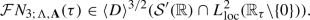(130)

Via Proposition 5.4, expanding the $$\textbf{k}$$th term in Eq. (107) in Taylor series around $$\tau = 2\pi |\textbf{k}|$$ and checking that the sum of the rest is smooth in a neighborhood of this point, and applying Proposition 5.3 with $$j=2,s=-3/2,p=2$$, this is not the case. $$\square$$

This completes the proof of Theorem 1.5.